The Australian Journal of Mathematical Analysis and Applications

 Home News Editors Volumes RGMIA Subscriptions Authors Contact

ISSN 1449-5910

Sorry death is imminent for file: ../public_html/searchroot/files/tex/v19n2/v19i2p4.tex
You searched for yu
Total of 254 results found in site

25: Paper Source PDF document

Paper's Title:

Ostrowski Type Inequalities for Lebesgue Integral: a Survey of Recent Results

Author(s):

Sever S. Dragomir1,2

1Mathematics, School of Engineering & Science
Victoria University, PO Box 14428
Melbourne City, MC 8001,
Australia
E-mail: sever.dragomir@vu.edu.au

2DST-NRF Centre of Excellence in the Mathematical and Statistical Sciences,
School of Computer Science & Applied Mathematics,
University of the Witwatersrand,
Private Bag 3, Johannesburg 2050,
South Africa
URL: http://rgmia.org/dragomir

Abstract:

The main aim of this survey is to present recent results concerning Ostrowski type inequalities for the Lebesgue integral of various classes of complex and real-valued functions. The survey is intended for use by both researchers in various fields of Classical and Modern Analysis and Mathematical Inequalities and their Applications, domains which have grown exponentially in the last decade, as well as by postgraduate students and scientists applying inequalities in their specific areas.

10: Paper Source PDF document

Paper's Title:

Oscillations of First Order Linear Delay Difference Equations

Author(s):

G. E. Chatzarakis and I. P. Stavroulakis

Department of Mathematics, University of Ioannina,
451 10, Greece
ipstav@cc.uoi.gr

Abstract:

Consider the first order linear delay difference equation of the form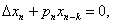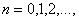where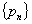is a sequence of nonnegative real numbers, k is a positive integer and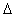denotes the forward difference operator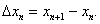New oscillation criteria are established when the well-known oscillation conditions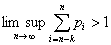and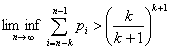are not satisfied. The results obtained essentially improve known results in the literature.

9: Paper Source PDF document

Paper's Title:

Integer Sums of Powers of Trigonometric Functions (MOD p), for prime p

Author(s):

G. J. Tee

Department of Mathematics, University of Auckland, Auckland,
New Zealand
tee@math.auckland.ac.nz

Abstract:

Many multi--parameter families of congruences (mod p) are found for integer sums of  qth powers of the trigonometric functions over various sets of equidistant arguments, where p is any prime factor of q. Those congruences provide sensitive tests for the accuracy of software for evaluating trigonometric functions to high precision.

6: Paper Source PDF document

Paper's Title:

A Low Order Least-Squares Nonconforming Finite Element Method for Steady Magnetohydrodynamic Equations

Author(s):

Z. Yu, D. Shi and H. Zhu

College of Science,
Zhongyuan University of Technology,
Zhengzhou 450007,
China.
E-mail: 5772@zut.edu.cn

School of Mathematics and Statistics,
Zhengzhou University,
Zhengzhou 450001,
China.
E-mail: shi_dy@126.com

Mathematics Department,
University of Southern Mississippi,
Hattiesburg MS, 39406,
U.S.A
E-mail: huiqing.zhu@usm.edu

Abstract:

A low order least-squares nonconforming finite element (NFE) method is proposed for magnetohydrodynamic equations with EQ1rot element and zero-order Raviart-Thomas element. Based on the above element's typical interpolations properties, the existence and uniqueness of the approximate solutions are proved and the optimal order error estimates for the corresponding variables are derived.

5: Paper Source PDF document

Paper's Title:

Essential Random Fixed Point Set of Random Operators

Author(s):

Ismat Beg

Centre for Advanced Studies in Mathematics,
Lahore University of Management Sciences (LUMS),
54792-Lahore, PAKISTAN. ibeg@lums.edu.pk
URL: http://web.lums.edu.pk/~ibeg

Abstract:

We obtain necessary and sufficient conditions for the existence of essential random fixed point of a random operator defined on a compact metric space. The structure of the set of essential random fixed points is also studied.

5: Paper Source PDF document

Paper's Title:

On the Generalized Inverse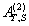over Integral Domains

Author(s):

Yaoming Yu and Guorong Wang

College of Education, Shanghai Normal University
Shanghai 200234
People's Republic of China.
yuyaoming@online.sh.cn
grwang@shnu.edu.cn

Abstract:

In this paper, we study further the generalized inverseof a matrix A over an integral domain. We give firstly some necessary and sufficient conditions for the existence of the generalized inverse, an explicit expression for the elements of the generalized inverseand an explicit expression for the generalized inverse, which reduces to the {1} inverse. Secondly, we verify that the group inverse, the Drazin inverse, the Moore-Penrose inverse and the weighted Moore-Penrose inverse are identical with the generalized inverse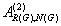for an appropriate matrix G, respectively, and then we unify the conditions for the existence and the expression for the elements of the weighted Moore-Penrose inverse, the Moore-Penrose inverse, the Drazin inverse and the group inverse over an integral domain. Thirdly, as a simple application, we give the relation between some rank equation and the existence of the generalized inverse, and a method to compute the generalized inverse. Finally, we give an example of evaluating the elements ofwithout calculating.

5: Paper Source PDF document

Paper's Title:

Isoperimetric Inequalities for Dual Harmonic Quermassintegrals

Author(s):

Yuan Jun, Zao Lingzhi and Duan Xibo

School of Mathematics and Computer Science,
Nanjing Normal University, Nanjing, 210097,
China.
yuanjun_math@126.com

Department of Mathematics, Nanjing Xiaozhuang University,
Nanjing, 211171,
China.
lzhzhao@163.com

Department of Mathematics, Shandong Water Polytechnic,
Shandong, 276826,
China
dxb1111@sohu.com

Abstract:

In this paper, some isoperimetric inequalities for the dual harmonic quermassintegrals are established.

5: Paper Source PDF document

Paper's Title:

A Geometric Generalization of Busemann-Petty Problem

Author(s):

Liu Rong and Yuan Jun

Shanghai Zhangjiang Group Junior Middle School,
China

Abstract:

The norm defined by Busemann's inequality establishes a class of star body - intersection body. This class of star body plays a key role in the solution of Busemann-Petty problem. In 2003, Giannapoulos  defined a norm for a new class of half-section. Based on this norm, we give a geometric generalization of Busemann-Petty problem, and get its answer as a result

4: Paper Source PDF document

Paper's Title:

Viability Theory And Differential Lanchester Type Models For Combat.
Differential Systems.

Author(s):

G. Isac and A. Gosselin

Department Of Mathematics, Royal Military College Of Canada,
P.O. Box 17000, S
tn Forces, Kingston, Ontario, Canada K7k 7b4

URL
:

URL
:

Abstract:

In 1914, F.W. Lanchester proposed several mathematical models based on differential equations to describe combat situations . Since then, his work has been extensively modified to represent a variety of competitions including entire wars. Differential Lanchester type models have been studied from many angles by many authors in hundreds of papers and reports. Lanchester type models are used in the planning of optimal strategies, supply and tactics. In this paper, we will show how these models can be studied from a viability theory stand point. We will introduce the notion of winning cone and show that it is a viable cone for these models. In the last part of our paper we will use the viability theory of differential equations to study Lanchester type models from the optimal theory point of view.

4: Paper Source PDF document

Paper's Title:

On the Optimal Buckling Loads of Clamped Columns

Author(s):

Samir Karaa

Department of Mathematics and Statistics
Sultan Qaboos University, P.O. Box 36, Alkhod 123
Muscat, Sultanate of Oman
skaraa@squ.edu.om

URL: http://ajmaa.org/EditorsU/SKaraa.php

Abstract:

We consider the problem of determining the optimal shape of a clamped column of given length and volume, without minimum cross section constraints. We prove that the necessary condition of optimality derived by Olhoff and Rasmussen is sufficient when 0<α<1. The number alpha appears in Equation 2.1. For the case α =1 it is shown that the value 48 is optimal. We also determine the exact values of the optimal shape at the extremities, and take advantage of a robust nonlinear ordinary differential equation solver COLSYS to compute the optimal buckling load with a high accuracy.

4: Paper Source PDF document

Paper's Title:

On Stan Ulam and his Mathematics

Author(s):

Krzysztof Ciesielski and Themistocles M. Rassias

Mathematics Institute, Jagiellonian University,
Ł
jasiewicza 6, 30-348 Kraków,
Poland
Department of Mathematics. National Technical University of Athens,
Zografou Campus, 15780 Athens,
Greece

Krzysztof.Ciesielski@im.uj.edu.pl
trassias@math.ntua.gr

Abstract:

In this note we give a glimpse of the curriculum vitae of Stan Ulam, his personality and some of the mathematics he was involved in.

4: Paper Source PDF document

Paper's Title:

Topological Aspects of Scalarization in Vector Optimization Problems.

Author(s):

Peter I. Kogut, Rosanna Manzo and Igor V. Nechay

Department of Differential Equations,
Dnipropetrovsk National University, Naukova STR.,
13, 49010 Dnipropetrovsk,
Ukraine

p.kogut@i.ua

Universitŕ di Salerno,
Dipartimento di Ingegneria dell'Informazione e Matematica Applicata,
Via Ponte don Melillo, 84084 Fisciano (SA),
Italy

manzo@diima.unisa.it

Department of Technical Cybernetics,
Dnipropetrovsk Technical University,
Acad. Lazarjan STR., 2, 49010 Dnipropetrovsk,
Ukraine

i.nechay@i.ua

Abstract:

In this paper, we study vector optimization problems in partially ordered Banach spaces. We suppose that the objective mapping possesses a weakened property of lower semicontinuity and make no assumptions on the interior of the ordering cone. We derive sufficient conditions for existence of efficient solutions of the above problems and discuss the role of topological properties of the objective space. We discuss the scalarization of vector optimization problems when the objective functions are vector-valued mappings with a weakened property of lower semicontinuity. We also prove the existence of the so-called generalized efficient solutions via the scalarization process. All principal notions and assertions are illustrated by numerous examples.

4: Paper Source PDF document

Paper's Title:

On Some Relations Among the Solutions of the Linear Volterra Integral Equations

Author(s):

Ismet Ozdemir and Faruk Temizer

Inönü Üniversitesi Eğitim Fakültesi,
44280-Malatya,
Turkey

ismet.ozdemir@inonu.edu.tr

omer.temizer@inonu.edu.tr

Abstract:

The sufficient conditions for y1(x)≤ y2(x) were given in  such that ym(x)=fm(x)+∫ax Km(x, t)ym(t)dt,(m=1,2) and x∈ [a, b]. Some properties such as positivity, boundedness and monotonicity of the solution of the linear Volterra integral equation of the form f(t)=1-∫0tK(t-τ)f(τ)dτ=1-K*f, (0≤ t<∞) were obtained, without solving this equation, in [3,4,5,6]. Also, the boundaries for functions f', f'',..., f(n),(n ∈ N) defined on the infinite interval [0, ∞) were found in [7,8].

In this work, for the given equation f(t)=1-K* f and n≥ 2, it is derived that there exist the functions  L2, L3,..., Ln which can be obtained by means of K and some inequalities among the functions f, h2, h3,..., hi for i=2, 3,...., n are satisfied on the infinite interval [0, ∞), where hi is the solution of the equation hi(t)=1-Li* hi and n is a natural number.

4: Paper Source PDF document

Paper's Title:

On a Class of Meromorphic Functions of Janowski Type Related with a Convolution Operator

Author(s):

Abdul Rahman S. Juma, Husamaldin I. Dhayea

Department of Mathematics,
Iraq.
E-mail: dr_juma@hotmail.com

Department of Mathematics,
Tikrit University, Tikrit,
Iraq.

Abstract:

In this paper, we have introduced and studied new operator \$Qkλ,m,γ by the Hadamard product (or convolution) of two linear operators Dkλ and Im,γ, then using this operator to study and investigate a new subclass of meromorphic functions of Janowski type, giving the coefficient bounds, a sufficient condition for a function to belong to the considered class and also a convolution property. The results presented provide generalizations of results given in earlier works.

4: Paper Source PDF document

Paper's Title:

The Dynamics of an Ebola Epidemic Model with Quarantine of Infectives

Author(s):

Eliab Horub Kweyunga

Department of Mathematics,
Kabale University,
P.O.Box 317, Kabale,
Uganda.

E-mail: hkweyunga@kab.ac.ug

Abstract:

The recurrent outbreaks of ebola in Africa present global health challenges. Ebola is a severe, very fatal disease with case fatality rates of up to 90%. In this paper, a theoretical deterministic model for ebola epidemic with quarantine of infectives is proposed and analyzed. The model exhibits two equilibria; the disease free and endemic equilibrium points. The basic reproduction number, R0, which is the main threshold, is obtained and the stability of the equilibrium points established. Using parameter values drawn from the 2014 West Africa ebola outbreak, a numerical simulation of the model is carried out. It is found that the dynamics of the model are completely determined by R0 and that a quarantine success rate of at least 70% is sufficient to contain the disease outbreak.

4: Paper Source PDF document

Paper's Title:

The Concept of Convergence for 2-Dimensional Subspaces Sequence in Normed Spaces

Author(s):

M. Manuharawati, D. N. Yunianti, M. Jakfar

Mathematics Department, Universitas Negeri Surabaya,
Jalan Ketintang Gedung C8,
Surabaya 60321,
Indonesia.
E-mail: manuharawati@unesa.ac.id, dwiyunianti@unesa.ac.id,

Abstract:

In this paper, we present a concept of convergence of sequence, especially, of 2-dimensional subspaces of normed spaces. The properties of the concept are established. As consequences of our definition in an inner product space, we also obtain the continuity property of the angle between two 2-dimensional subspaces of inner product spaces.

4: Paper Source PDF document

Paper's Title:

A Fuzzy Soft Quotient Topology and Its Properties

Author(s):

Haripamyu, Riri Alfakhriati, Monika Rianti Helmi, Jenizon

Department of Mathematics and Data Science, Andalas University, Padang, Indonesia.
E-mail: haripamyu@sci.unand.ac.id
ririalfakhriati123@gmail.com
monikariantihelmi@sci.unand.ac.id
jenizon@gmail.com

Abstract:

This research is to construct a new topology on fuzzy soft set by using the concept of quotient topology. Then we study the concept of quotient map to define the fuzzy soft quotient map and provide some relevant properties of fuzzy soft quotient map. Furthermore, we give some examples related to fuzzy soft quotient topology and fuzzy soft quotient map to apply some properties of fuzzy soft quotient map.

3: Paper Source PDF document

Paper's Title:

Analytical and Numerical Solutions of the Inhomogenous Wave Equation

Author(s):

T. Matsuura and S. Saitoh

Department of Mechanical Engineering, Faculty of Engineering,
Gunma University, Kiryu 376-8515, Japan
matsuura@me.gunma-u.ac.jp

Department of Mathematics, Faculty of Engineering,
Gunma University, Kiryu 376-8515, Japan
ssaitoh@math.sci.gunma-u.ac.jp

Abstract:

In this paper, by a new concept and method we give approximate solutions of the inhomogenous wave equation on multidimensional spaces. Numerical experiments are conducted as well.

3: Paper Source PDF document

Paper's Title:

Norm Estimates for the Difference between Bochner’s Integral and the Convex Combination of Function’s Values

Author(s):

P. Cerone, Y.J. Cho, S.S. Dragomir, J.K. Kim, and S.S. Kim

School of Computer Science and Mathematics,
Victoria University of Technology,
Po Box 14428, Mcmc 8001, Victoria, Australia.

URL
:

Department of Mathematics Education, College of Education,
Gyeongsang National University, Chinju 660-701, Korea

School of Computer Science and Mathematics,
Victoria University of Technology,
Po Box 14428, Mcmc 8001, Victoria, Australia.

URL
:

Department of Mathematics, Kyungnam University,
Masan,, Kyungnam 631-701, Korea

Department of Mathematics, Dongeui University,
Pusan 614-714, Korea

Abstract:

Norm estimates are developed between the Bochner integral of a vector-valued function in Banach spaces having the Radon-Nikodym property and the convex combination of function values taken on a division of the interval [a, b].

3: Paper Source PDF document

Paper's Title:

On Pseudo Almost Periodic Solutions to Some Neutral Functional-Differential Equations

Author(s):

Toka Diagana and Eduardo Hernández

Department of Mathematics, Howard University
2441 6th Street NW, Washington DC 20059,
USA.
tdiagana@howard.edu

Departamento de Matemática, I.C.M.C. Universidade de Săo Paulo,
Caixa Postal 668, 13560-970, Săo Carlos SP,
Brazil.
lalohm@icmc.sc.usp.br

Abstract:

This paper discusses the existence and uniqueness of pseudo almost periodic solutions to a class of partial neutral functional-differential equations. Under some suitable assumptions, existence and uniqueness results are obtained. An example is given to illustrate abstract results.

3: Paper Source PDF document

Paper's Title:

Existence Results for Perturbed Fractional Differential Inclusions

Author(s):

Y.-K. Chang

Department of Mathematics,
Lanzhou Jiaotong University, Lanzhou, Gansu 730070, People's
Republic of China
lzchangyk@163.com

Abstract:

This paper is mainly concerned with the following fractional differential inclusions with boundary condition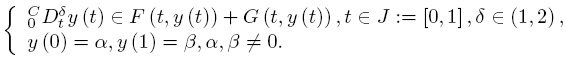A sufficient condition is established for the existence of solutions of the above problem by using a fixed point theorem for multivalued maps due to Dhage. Our result is proved under the mixed generalized Lipschitz and Carathéodory conditions.

3: Paper Source PDF document

Paper's Title:

A Short Proof of an Open Inequality with Power-Exponential Functions

Author(s):

Mitsuhiro Miyagi and Yusuke Nishizawa

General Education, Ube National College of Technology,
Yamaguchi 755-8555,
Japan

E-mail: miyagi@ube-k.ac.jp
yusuke@ube-k.ac.jp

Abstract:

V. Cîrtoaje conjectured that a3b + b3a + ( (a -b)/2 )4 ≤ 2 holds for all nonnegative numbers a and b with a +b =2. In this short note, we give a proof of the Cîrtoaje's conjecture with power-exponential functions.

3: Paper Source PDF document

Paper's Title:

The Effect of Harvesting Activities on Prey-Predator Fishery Model with Holling type II in Toxicant Aquatic Ecosystem

Author(s):

Moh Nurul Huda, Fidia Deny Tisna Amijaya, Ika Purnamasari

Department of Mathematics, Faculty of Mathematics and Natural Science,
Mulawarman University,
Samarinda, East Kalimantan,75123
Indonesia.
E-mail: muh.nurulhuda@fmipa.unmul.ac.id
ika.purnamasari@fmipa.unmul.ac.id

Abstract:

This paper discussed prey-predator fishery models, in particular by analysing the effects of toxic substances on aquatic ecosystems. It is assumed in this model, that the prey population is plankton and the predator population is fish.\ Interaction between the two populations uses the Holling type II function. The existence of local and global critical points of the system are shown and their stability properties are analysed. Furthermore, Bionomic equilibrium and optimal control of harvesting are discussed. Finally, numerical simulations have been carried out to show in the interpretation of results.

3: Paper Source PDF document

Paper's Title:

Riemann-Stieltjes Integrals and Some Ostrowski Type Inequalities

Author(s):

W. G. Alshanti

Department of General Studies,
Jubail University College,
KSA.
E-mail: shantiw@ucj.edu.sa

Abstract:

In this article, we investigate new integral inequalities of Ostrowski's type of various functional aspects. For mapping's second derivative, we assume two cases, namely, L1 and L spaces. Moreover, for first derivative, we investigate two different characteristics, namely, bounded variation and locally Lipchitz continuity. Applications to special means and composite quadrature rules are also carried out.

3: Paper Source PDF document

Paper's Title:

An Integration Technique for Evaluating Quadratic Harmonic Sums

Author(s):

J. M. Campbell and K.-W. Chen

Department of Mathematics and Statistics,
York University, 4700 Keele St, Toronto,
ON M3J 1P3,
E-mail: jmaxwellcampbell@gmail.com

Department of Mathematics, University of Taipei,
Taipei 10048, Taiwan.
E-mail: kwchen@uTaipei.edu.tw
URL: https://math.utaipei.edu.tw/p/412-1082-22.php

Abstract:

The modified Abel lemma on summation by parts has been applied in many ways recently to determine closed-form evaluations for infinite series involving generalized harmonic numbers with an upper parameter of two. We build upon such results using an integration technique that we apply to ``convert'' a given evaluation for such a series into an evaluation for a corresponding series involving squared harmonic numbers.

2: Paper Source PDF document

Paper's Title:

On Vector Variational Inequality Problem in Terms of Bifunctions

Author(s):

C. S. Lalitha and Monika Mehta

Department of Mathematics, Rajdhani College,
University of Delhi, Raja Garden,
Delhi 110015, India
cslalitha@rediffmail.com

Department of Mathematics, Satyawati College,
University Of Delhi, Ashok Vihar,
Phase-III, Delhi 110052, India
mridul_in@yahoo.com

Abstract:

In this paper, we consider a generalized vector variational inequality problem expressed in terms of a bifunction and establish existence theorems for this problem by using the concepts of cone convexity and cone strong quasiconvexity and employing the celebrated Fan's Lemma. We also give two types of gap functions for this problem.

2: Paper Source PDF document

Paper's Title:

Local and Global Existence and Uniqueness Results for Second and Higher Order Impulsive Functional Differential Equations with Infinite Delay

Author(s):

Johnny Henderson and Abdelghani Ouahab

Department of Mathematics, Baylor University,
Waco, Texas 76798-7328
USA.
Johnny_Henderson@baylor.edu

Laboratoire de Mathématiques, Université de Sidi Bel Abbés
BP 89, 22000 Sidi Bel Abbées,
Algérie.
ouahab@univ-sba.dz

Abstract:

In this paper, we discuss the local and global existence and uniqueness results for second and higher order impulsive functional differential equations with infinite delay. We shall rely on a nonlinear alternative of Leray-Schauder. For the global existence and uniqueness we apply a recent Frigon and Granas nonlinear alternative of Leray-Schauder type in Fréchet spaces.

2: Paper Source PDF document

Paper's Title:

Existence of Solutions for Third Order Nonlinear Boundary Value Problems

Author(s):

Yue Hu and Zuodong Yang

School of Mathematics and Computer Science, Nanjing Normal University, Jiangsu Nanjing 210097,
China.
huu3y2@163.com

College of Zhongbei, Nanjing Normal University, Jiangsu Nanjing 210046,
China.
zdyang_jin@263.net
yangzuodong@njnu.edu.cn

Abstract:

In this paper, the existence of solution for a class of third order quasilinear ordinary differential equations with nonlinear boundary value problems

p(u"))'=f(t,u,u',u"), u(0)=A, u'(0)=B, R(u'(1),u"(1))=0

is established. The results are obtained by using upper and lower solution methods.

2: Paper Source PDF document

Paper's Title:

The Convergence of Modified Mann-Ishikawa Iterations when Applied to an Asymptotically Pseudocontractive Map

Author(s):

S. Soltuz

Departamento de Matematicas, Universidad de Los Andes, Carrera 1
No. 18A-10, Bogota,
Colombia
and
``T. Popoviciu" Institute of Numerical Analysis
Cluj-Napoca,
Romania
smsoltuz@gmail.com
URL:http://www.uniandes.edu.co/

Abstract:

We prove that under minimal conditions the modified Mann and Ishikawa iterations converge when dealing with an asymptotically pseudocontractive map. We give an affirmative answer to the open question from C.E. Chidume and H. Zegeye, Approximate fixed point sequences and convergence theorems for asymptotically pseudocontractive mappings, J. Math. Anal. Appl., 278 (2003), 354--366.

2: Paper Source PDF document

Paper's Title:

On a Subclass of Uniformly Convex Functions Defined by the Dziok-Srivastava Operator

Author(s):

M. K. Aouf and G. Murugusundaramoorthy

Mathematics Department, Faculty of Science,
Mansoura University 35516,
Egypt.
mkaouf127@yahoo.com

School of Science and Humanities, VIT University
Vellore - 632014,
India.
gmsmoorthy@yahoo.com

Abstract:

Making use of the Dziok-Srivastava operator, we define a new subclass Tlm([α1];α,β) of uniformly convex function with negative coefficients. In this paper, we obtain coefficient estimates, distortion theorems, locate extreme points and obtain radii of close-to-convexity, starlikeness and convexity for functions belonging to the class Tlm([α1];α,β) . We consider integral operators associated with functions belonging to the class Hlm([α1];α,β) defined via the Dziok-Srivastava operator. We also obtain several results for the modified Hadamard products of functions belonging to the class Tlm([α1];α,β) and we obtain properties associated with generalized fractional calculus operators.

2: Paper Source PDF document

Paper's Title:

Applications of Relations and Relators in the Extensions of Stability Theorems for Homogeneous and Additive Functions

Author(s):

Árpád Száz

Institute of Mathematics, University of Debrecen,
H-4010 Debrecen, Pf. 12,
Hungary
szaz@math.klte.hu

Abstract:

By working out an appropriate technique of relations and relators and extending the ideas of the direct methods of Z. Gajda and R. Ger, we prove some generalizations of the stability theorems of D. H. Hyers, T. Aoki, Th. M. Rassias and P. Găvruţă in terms of the existence and unicity of 2-homogeneous and additive approximate selections of generalized subadditive relations of semigroups to vector relator spaces. Thus, we obtain generalizations not only of the selection theorems of Z. Gajda and R. Ger, but also those of the present author.

2: Paper Source PDF document

Paper's Title:

Ellipses of Minimal Area and of Minimal Eccentricity Circumscribed About a Convex Quadrilateral

Author(s):

Alan Horwitz

Penn State University,
25 Yearsley Mill Rd.,
Media, PA 19063,
U.S.A
alh4@psu.edu

Abstract:

First, we fill in key gaps in Steiner's nice characterization of the most nearly circular ellipse which passes through the vertices of a convex quadrilateral,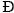. Steiner proved that there is only one pair of conjugate directions, M1 and M2, that belong to all ellipses of circumscription. Then he proves that if there is an ellipse, E, whose equal conjugate diameters possess the directional constants M1 and M2, then E must be an ellipse of circumscription which has minimal eccentricity. However, Steiner does not show the existence or uniqueness of such an ellipse. We prove that there is a unique ellipse of minimal eccentricity which passes through the vertices of. We also show that there exists an ellipse which passes through the vertices ofand whose equal conjugate diameters possess the directional constants M1 and M2. We also show that there exists a unique ellipse of minimal area which passes through the vertices of. Finally, we call a convex quadrilateral,, bielliptic if the unique inscribed and circumscribed ellipses of minimal eccentricity have the same eccentricity. This generalizes the notion of bicentric quadrilaterals. In particular, we show the existence of a bielliptic convex quadrilateral which is not bicentric.

2: Paper Source PDF document

Paper's Title:

Generalized Efficient Solutions to One Class of Vector Optimization Problems in Banach Space

Author(s):

Peter I. Kogut, Rosanna Manzo, and Igor V. Nechay

Department of Differential Equations,
Dnipropetrovsk National University,
Naukova str., 13, 49050 Dnipropetrovsk,
Ukraine
p.kogut@i.ua

Dipartimento di Ingegneria Dell’informazione e Matematica Applicata,
Universitŕ di Salerno,
Via Ponte Don Melillo, 84084 Fisciano (Sa),
Italy
manzo@diima.unisa.it

Department of Technical Cybernetics,
Dnipropetrovsk Technical University,
Dnipro
petrovsk,
Ukraine
i.nechay@i.ua

Abstract:

In this paper, we study vector optimization problems in Banach spaces for essentially nonlinear operator equations with additional control and state constraints. We assume that an objective mapping possesses a weakened property of lower semicontinuity and make no assumptions on the interior of the ordering cone. Using the penalization approach we derive both sufficient and necessary conditions for the existence of efficient solutions of the above problems. We also prove the existence of the so-called generalized efficient solutions via the scalarization of some penalized vector optimization problem.

2: Paper Source PDF document

Paper's Title:

Approximation of Common Fixed Points of a Finite Family of Asymptotically Demicontractive Mappings in Banach Spaces

Author(s):

Yuchao Tang, Yong Cai, Liqun Hu and Liwei Liu

Department of Mathematics, NanChang University,
Nanchang 330031, P.R. China
Department of Mathematics, Xi'an Jiaotong University,
Xi'an 710049, P.R. China

hhaaoo1331@yahoo.com.cn

Abstract:

By virtue of new analytic techniques, we analyze and study
several strong convergence theorems for the approximation of
common fixed points of asymptotically demicontractive mappings
via the multistep iterative sequence with errors in Banach
spaces. Our results improve and extend the corresponding ones
announced by Osilike , Osilike and Aniagbosor, Igbokwe, Cho et
al., Moore and Nnoli, Hu and all the others.

2: Paper Source PDF document

Paper's Title:

Necessary and Sufficient Conditions for Cyclic Homogeneous Polynomial Inequalities of Degree Four in Real Variables

Author(s):

Vasile Cirtoaje and Yuanzhe Zhou

Department of Automatic Control and Computers
University of Ploiesti
Romania.
vcirtoaje@upg-ploiesti.ro.

High School Affiliated to Wuhan University, China

Abstract:

In this paper, we give two sets of necessary and sufficient conditions that the inequality f4(x,y,z) ≥ 0 holds for any real numbers x,y,z, where f4(x,y,z) is a cyclic homogeneous polynomial of degree four. In addition, all equality cases of this inequality are analysed. For the particular case in which f4(1,1,1)=0, we get the main result in . Several applications are given to show the effectiveness of the proposed methods.

2: Paper Source PDF document

Paper's Title:

Asymptotic Analysis of Positive Decreasing Solutions of a Class of Systems of Second Order Nonlinear Differential Equations in the Framework of Regular Variation

Author(s):

Jaroslav Jaroš, Kusano Takaŝi, Tomoyuki Tanigawa

Department of Mathematical Analysis and Numerical Mathematics,
Faculty of Mathematics, Physics and Informatics,
Comenius Universiy, 842 48 Bratislava,
Slovakia.

E-mail: ksntksjm4@gmail.com

Professor Emeritus at: Hiroshima University,
Department of Mathematics, Faculty of Science,
Higashi-Hiroshima 739-8526,
Japan.

E-mail: jaros@fmph.uniba.sk

Department of Mathematics, Faculty of Education,
Kumamoto University, Kumamoto 860-8555,
Japan.

E-mail: tanigawa@educ.kumamoto-u.ac.jp

Abstract:

The system of nonlinear differential equations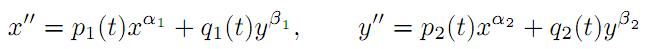is under consideration, where αi and βi are positive constants and pi(t) and qi(t) are continuous regularly varying functions on [a,). Two kinds of criteria are established for the existence of strongly decreasing regularly varying solutions with negative indices of (A) with precise asymptotic behavior at infinity. Fixed point techniques and basic theory of regular variation are utilized for this purpose.

2: Paper Source PDF document

Paper's Title:

Inequalities for Discrete F-Divergence Measures: A Survey of Recent Results

Author(s):

Sever S. Dragomir1,2

1Mathematics, School of Engineering & Science
Victoria University, PO Box 14428
Melbourne City, MC 8001,
Australia
E-mail: sever.dragomir@vu.edu.au

2DST-NRF Centre of Excellence in the Mathematical and Statistical Sciences,
School of Computer Science & Applied Mathematics,
University of the Witwatersrand,
Private Bag 3, Johannesburg 2050,
South Africa
URL: http://rgmia.org/dragomir

Abstract:

In this paper we survey some recent results obtained by the author in providing various bounds for the celebrated f-divergence measure for various classes of functions f. Several techniques including inequalities of Jensen and Slater types for convex functions are employed. Bounds in terms of Kullback-Leibler Distance, Hellinger Discrimination and Varation distance are provided. Approximations of the f-divergence measure by the use of the celebrated Ostrowski and Trapezoid inequalities are obtained. More accurate approximation formulae that make use of Taylor's expansion with integral remainder are also surveyed. A comprehensive list of recent papers by several authors related this important concept in information theory is also included as an appendix to the main text.

2: Paper Source PDF document

Paper's Title:

Integrating Factors and First Integrals of a Class of Third Order Differential Equations

Author(s):

Department of Mathematics,
Yarmouk University,
Irbid 21163,
Jordan.

Abstract:

The principle of finding an integrating factor for a none exact differential equations is extended to a class of third order differential equations. If the third order equation is not exact, under certain conditions, an integrating factor exists which transforms it to an exact one. Hence, it can be reduced into a second order differential equation. In this paper, we give explicit forms for certain integrating factors of a class of the third order differential equations.

2: Paper Source PDF document

Paper's Title:

A Method of the Study of the Cauchy Problem for~a~Singularly Perturbed Linear Inhomogeneous Differential Equation

Author(s):

E. E. Bukzhalev and A. V. Ovchinnikov

Faculty of Physics,
Moscow State University,
1 Leninskie Gory,
Moscow, 119991,
Russia
E-mail: bukzhalev@mail.ru

Russian Institute for Scientific and Technical Information of the Russian Academy of Sciences,
20 Usievicha St., Moscow, 125190,
Russia
E-mail: ovchinnikov@viniti.ru

Abstract:

We construct a sequence that converges to a solution of the Cauchy problem for a singularly perturbed linear inhomogeneous differential equation of an arbitrary order. This sequence is also an asymptotic sequence in the following sense: the deviation (in the norm of the space of continuous functions) of its nth element from the solution of the problem is proportional to the (n+1)th power of the parameter of perturbation. This sequence can be used for justification of asymptotics obtained by the method of boundary functions.

2: Paper Source PDF document

Paper's Title:

On Finding Integrating Factors and First Integrals for a Class of Higher Order Differential Equations

Author(s):

Department of Mathematics,
Yarmouk University,
Irbid, 21163,
Jordan.

Abstract:

If the \$n-th\$ order differential equation is not exact, under certain conditions, an integrating factor exists which transforms the differential equation into an exact one. Thus, the order of differential equation can be reduced to the lower order. In this paper, we present a technique for finding integrating factors of the following class of differential equations: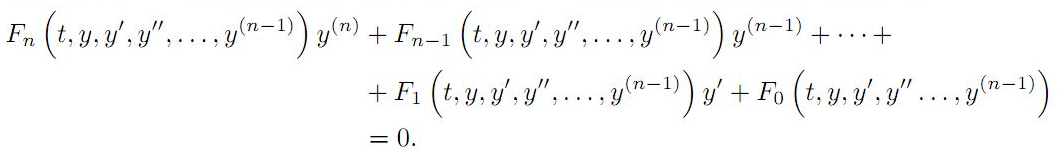Here, the functions F0,F1,F2, ,Fn are assumed to be continuous functions with their first partial derivatives on some simply connected domain Ω Rn+1. We also presented some demonstrative examples

2: Paper Source PDF document

Paper's Title:

On a New Class of Eulerian's Type Integrals Involving Generalized Hypergeometric Functions

Author(s):

Sungtae Jun, Insuk Kim and Arjun K. Rathie

General Education Institute,
Konkuk University, Chungju 380-701,
Republic of Korea.

Department of Mathematics Education,
Wonkwang University, Iksan, 570-749,
Republic of Korea.

Department of Mathematics,
Vedant College of Engineering and Technology (Rajasthan Technical University),
Bundi-323021, Rajasthan,
India.

E-mail: sjun@kku.ac.kr, iki@wku.ac.kr, arjunkumarrathie@gmail.com

Abstract:

Very recently Masjed-Jamei and Koepf established interesting and useful generalizations of various classical summation theorems for the 2F1, 3F2, 4F3, 5F4 and 6F5 generalized hypergeometric series. The main aim of this paper is to establish eleven Eulerian's type integrals involving generalized hypergeometric functions by employing these theorems. Several special cases have also been given.

2: Paper Source PDF document

Paper's Title:

On an extension of Edwards's double integral with applications

Author(s):

I. Kim, S. Jun, Y. Vyas and A. K. Rathie

Department of Mathematics Education,
Wonkwang University,
Iksan, 570-749,
Republic of Korea.

General Education Institute,
Konkuk University,
Chungju 380-701,
Republic of Korea.

Department of Mathematics, School of Engineering,
Bhatewar, Udaipur, 313601, Rajasthan State,
India.

Department of Mathematics,
Vedant College of Engineering and Technology,
(Rajasthan Technical University),
Bundi-323021, Rajasthan,
India.

E-mail: iki@wku.ac.kr sjun@kku.ac.kr
yashoverdhan.vyas@spsu.ac.in arjunkumarrathie@gmail.com

Abstract:

The aim of this note is to provide an extension of the well known and useful Edwards's double integral. As an application, new class of twelve double integrals involving hypergeometric function have been evaluated in terms of gamma function. The results are established with the help of classical summation theorems for the series 3F2 due to Watson, Dixon and Whipple. Several new and interesting integrals have also been obtained from our main findings.

2: Paper Source PDF document

Paper's Title:

On the Polyconvolution of Hartley Integral Transforms H1, H2, H1 and Integral Equations

Author(s):

Nguyen Minh Khoa and Dau Xuan Luong

Department of Mathematics,
Electric Power University,
Ha Noi, and Faculty of Fundamental Science,
Ha Long University, Quang Ninh,
Viet Nam.
E-mail: khoanm@epu.edu.vn, dauxuanluong@gmail.com

Abstract:

In this paper, we construct and study a new polyconvolution * (f,g,h)(x) of functions f, g, h. We will show that the polyconvolution satisfy the following factorization equality

H1[*(f,g,h)](y)=(H2f)(y)(H1g)(y)(H1h)(y), y R

We prove the existence of this polyconvolution in the space L(R). As examples, applications to solve an integral equation of polyconvolution type and two systems of integral equations of polyconvolution type are presented.

2: Paper Source PDF document

Paper's Title:

Bounds on the Jensen Gap, and Implications for Mean-Concentrated Distributions

Author(s):

Xiang Gao, Meera Sitharam, Adrian E. Roitberg

Department of Chemistry, and Department of Computer & Information Science & Engineering,
University of Florida,
Gainesville, FL 32611,
USA.
E-mail: qasdfgtyuiop@gmail.com

Abstract:

This paper gives upper and lower bounds on the gap in Jensen's inequality, i.e., the difference between the expected value of a function of a random variable and the value of the function at the expected value of the random variable. The bounds depend only on growth properties of the function and specific moments of the random variable. The bounds are particularly useful for distributions that are concentrated around the mean, a commonly occurring scenario such as the average of i.i.d. samples and in statistical mechanics.

2: Paper Source PDF document

Paper's Title:

Some fixed point results in partial S-metric spaces

Author(s):

M. M. Rezaee, S. Sedghi, A. Mukheimer, K. Abodayeh, and Z. D. Mitrovic

Department of Mathematics, Qaemshahr Branch,
Iran.

Department of Mathematics, Qaemshahr Branch,
Iran.
E-mail: sedghi.gh@qaemiau.ac.ir

Department of Mathematics and General Sciences,
KSA.
E-mail: mukheimer@psu.edu.sa

Department of Mathematics and General Sciences,
KSA.
E-mail: kamal@psu.edu.sa

Nonlinear Analysis Research Group,
Faculty of Mathematics and Statistics,
Ton Duc Thang University, Ho Chi Minh City,
Vietnam.
E-mail: zoran.mitrovic@tdtu.edu.vn

Abstract:

We introduce in this article a new class of generalized metric spaces, called partial S-metric spaces. In addition, we also give some interesting results on fixed points in the partial S-metric spaces and some applications.

2: Paper Source PDF document

Paper's Title:

Numerical Approximation by the Method of Lines with Finite-volume Approach of a Solute Transport Equation in Periodic Heterogeneous Porous Medium

Author(s):

D. J. Bambi Pemba and B. Ondami

Université Marien Ngouabi,
Factuté des Sciences et Techniques,
BP 69, Brazzaville,
Congo.
E-mail: bondami@gmail.com

Abstract:

In this paper we are interested in the numerical approximation of a two-dimensional solute transport equation in heterogeneous porous media having periodic structures. It is a class of problems which has been the subject of various works in the literature, where different methods are proposed for the determination of the so-called homogenized problem. We are interested in this paper, in the direct resolution of the problem, and we use the method of lines with a finite volume approach to discretize this equation. This discretization leads to an ordinary differential equation (ODE) that we discretize by the Euler implicit scheme. Numerical experiments comparing the obtained solution and the homogenized problem solution are presented. They show that the precision and robustness of this method depend on the ratio between, the mesh size and the parameter involved in the periodic homogenization.

2: Paper Source PDF document

Paper's Title:

Solving Non-Autonomous Nonlinear Systems of Ordinary Differential Equations Using Multi-Stage Differential Transform Method

Author(s):

K. A. Ahmad, Z. Zainuddin, F. A. Abdullah

School of Mathematical Sciences Universiti Sains Malaysia
11800 USM Penang
Malaysia.
zarita@usm.my
farahaini@usm.my

Abstract:

Differential equations are basic tools to describe a wide variety of phenomena in nature such as, electrostatics, physics, chemistry, economics, etc. In this paper, a technique is developed to solve nonlinear and linear systems of ordinary differential equations based on the standard Differential Transform Method (DTM) and Multi-stage Differential Transform Method (MsDTM). Comparative numerical results that we are obtained by MsDTM and Runge-Kutta method are proposed. The numerical results showed that the MsDTM gives more accurate approximation as compared to the Runge-Kutta numerical method for the solutions of nonlinear and linear systems of ordinary differential equations

2: Paper Source PDF document

Paper's Title:

Inequalities of Gamma Function Appearing in Generalizing Probability Sampling Design

Author(s):

Department of Mathematics,
Yarmouk University,
Irbid 21163,
Jordan.

Department of Statistics,
Yarmouk University,
Irbid 21163,
Jordan.

Abstract:

In this paper, we investigate the complete monotonicity of some functions involving gamma function. Using the monotonic properties of these functions, we derived some inequalities involving gamma and beta functions. Such inequalities can be used to generalize different probability distribution functions. Also, they can be used to generalize some statistical designs, e.g., the probability proportional to the size without replacement design.

2: Paper Source PDF document

Paper's Title:

Rational Expressions of Arithmetic and Geometric Means for the Sequence npn ∈ N and the Geometric Progression

Author(s):

M. Kinegawa, S. Miyamoto and Y. Nishizawa

Faculty of Education, Saitama University,
Shimo-okubo 255, Sakura-ku, Saitama-city, Saitama,
Japan.
E-mail: m.kinegawa.645@ms.saitama-u.ac.jp

Faculty of Education, Saitama University,
Shimo-okubo 255, Sakura-ku, Saitama-city, Saitama,
Japan.
E-mail: s.miyamoto.245@ms.saitama-u.ac.jp

Faculty of Education, Saitama University,
Shimo-okubo 255, Sakura-ku, Saitama-city, Saitama,
Japan.
E-mail: ynishizawa@mail.saitama-u.ac.jp

Abstract:

In this paper, we consider the arithmetic and geometric means for the sequence npn ∈ N and the geometric progression. We obtain the results associated with the rational expressions of the means.

2: Paper Source PDF document

Paper's Title:

Evaluation of a New Class of Double Integrals Involving Generalized Hypergeometric Function 4F3

Author(s):

Joohyung Kim, Insuk Kim and Harsh V. Harsh

Department of Mathematics Education,
Wonkwang University, Iksan, 570-749,
Korea.
E-mail: joohyung@wku.ac.kr

Department of Mathematics Education,
Wonkwang University, Iksan, 570-749,
Korea.
E-mail: iki@wku.ac.kr

Department of Mathematics, Amity School of Eng. and Tech.,
Amity University Rajasthan
NH-11C, Jaipur-303002, Rajasthan,
India.
E-mail: harshvardhanharsh@gmail.com

Abstract:

Very recently, Kim evaluated some double integrals involving a generalized hypergeometric function 3F2 with the help of generalization of Edwards's well-known double integral due to Kim,  et al. and generalized classical Watson's summation theorem obtained earlier by Lavoie,  et al. In this research paper we evaluate one hundred double integrals involving generalized hypergeometric function 4F3 in the form of four master formulas (25 each) viz. in the most general form for any integer. Some interesting results have also be obtained as special cases of our main findings.

2: Paper Source PDF document

Paper's Title:

Oscillatory Behavior of Second-Order Non-Canonical Retarded Difference Equations

Author(s):

G.E. Chatzarakis1, N. Indrajith2, E. Thandapani3 and K.S. Vidhyaa4

1Department of Electrical and Electronic Engineering Educators,
School of Pedagogical and Technological Education,
Marousi 15122, Athens,
Greece.
E-mail:  gea.xatz@aspete.gr, geaxatz@otenet.gr

2Department of Mathematics,
Presidency College, Chennai - 600 005,
India.
E-mail: indrajithna@gmail.com

3Ramanujan Institute for Advanced Study in Mathematics,
Chennai - 600 005,
India.
E-mail: ethandapani@yahoo.co.in

4Department of Mathematics,
SRM Easwari Engineering College,
Chennai-600089,
India.
E-mail: vidyacertain@gmail.com

Abstract:

Using monotonic properties of nonoscillatory solutions, we obtain new oscillatory criteria for the second-order non-canonical difference equation with retarded argument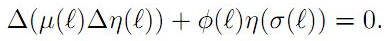Our oscillation results improve and extend the earlier ones. Examples illustrating the results are provided.

2: Paper Source PDF document

Paper's Title:

Preserver of Local Spectrum of Skew-product Operators

Author(s):

1Department of Mathematics,
College of Sciences,
University of Yasouj,
Yasouj, 75918-74934,
Iran.
E-mail: r.parvinian@yu.ac.ir

2Department of Mathematics,
College of Sciences,
University of Yasouj,
Yasouj, 75918-74934,
Iran.

3Department of Mathematics,
Ghor Institute of higher education,
Afghanistan.
E-mail: jumapazhman@gmail.com

Abstract:

Let H and K be infinite-dimensional complex Hilbert spaces, and B(H) (resp. B(K)) be the algebra of all bounded linear operators on H (resp. on K). For an operator T B(H) and a vector h H, let σT(h) denote the local spectrum of T at h. For two nonzero vectors h0 H and k0 K, we show that if two maps φ1 and φ2 from B(H) into B(K) satisfy

σφ1(T)φ2(S)*(k0)= σTS*(h0})

for all T, S B(H), and their range containing all operators of rank at most two, then there exist bijective linear maps P : H K and Q : K H such that φ1(T) = PTQ and φ2(T)* =Q-1T*P-1 for all T B(H). Also, we obtain some interesting results in this direction.

2: Paper Source PDF document

Paper's Title:

SQIRV Model for Omicron Variant with Time Delay

Author(s):

S. Dickson, S. Padmasekaran, G. E. Chatzarakis and S. L. Panetsos

Mathematics, Periyar University, Periyar Palkalai Nagar, Salem,
India.

Electrical and Electronic Engineering Educators, School of
Pedagogical and Technological Education (ASPETE),
Marousi 15122, Athens,
Greece.
E-mail: geaxatz@otenet.gr, spanetsos@aspete.gr

Abstract:

In order to examine the dynamics of the Omicron variant, this paper uses mathematical modelling and analysis of a SQIRV model, taking into account the delay in the conversion of susceptible individuals into infected individuals and infected individuals into recovered individuals. The pandemic was eventually controlled as a result of the massive delays. To assure the safety of the host population, this concept incorporates quarantine and the COVID-19 vaccine. Both local and global stability of the model are examined. It is found that the fundamental reproduction number affects both local and global stability conditions. Our findings show that asymptomatic cases caused by an affected population play an important role in increasing Omicron infection in the general population. The most recent data on the pandemic Omicron variant from Tamil Nadu, India, is verified.

2: Paper Source PDF document

Paper's Title:

Higher Order Accurate Compact Schemes for Time Dependent Linear and Nonlinear Convection-Diffusion Equations

Author(s):

S. Thomas, Gopika P.B. and S. K. Nadupuri

Department of Mathematics
National Institute of Technology Calicut
Kerala
673601
India.
E-mail: sobinputhiyaveettil@gmail.com pbgopika@gmail.com nsk@nitc.ac.in

Abstract:

The primary objective of this work is to study higher order compact finite difference schemes for finding the numerical solution of convection-diffusion equations which are widely used in engineering applications. The first part of this work is concerned with a higher order exponential scheme for solving unsteady one dimensional linear convection-diffusion equation. The scheme is set up with a fourth order compact exponential discretization for space and cubic \$C^1\$-spline collocation method for time. The scheme achieves fourth order accuracy in both temporal and spatial variables and is proved to be unconditionally stable. The second part explores the utility of a sixth order compact finite difference scheme in space and Huta's improved sixth order Runge-Kutta scheme in time combined to find the numerical solution of one dimensional nonlinear convection-diffusion equations. Numerical experiments are carried out with Burgers' equation to demonstrate the accuracy of the new scheme which is sixth order in both space and time. Also a sixth order in space predictor-corrector method is proposed. A comparative study is performed of the proposed schemes with existing predictor-corrector method. The investigation of computational order of convergence is presented.

1: Paper Source PDF document

Paper's Title:

New Reverses of Schwarz, Triangle and Bessel Inequalities in Inner Product Spaces

Author(s):

S. S. Dragomir

School of Computer Science and Mathematics, Victoria University of Technology, PO BOX
14428, MCMC 8001, VICTORIA, AUSTRALIA.

sever.dragomir@vu.edu.au
URL
: http://rgmia.vu.edu.au/SSDragomirWeb.html

Abstract:

New reverses of the Schwarz, triangle and Bessel inequalities in inner product spaces are pointed out. These results complement the recent ones obtained by the author in the earlier paper . Further, they are employed to establish new Grüss type inequalities. Finally, some natural integral inequalities are stated as well.

1: Paper Source PDF document

Paper's Title:

On an Extension of Hilbert’s Integral Inequality with Some Parameters

Author(s):

Bicheng Yang

Department of Mathematics, Guangdong Education College, Guangzhou, Guangdong 510303, People’s Republic of China.
URL
:

Abstract:

In this paper, by introducing some parameters and estimating the weight function, we give an extension of Hilbert’s integral inequality with a best constant factor. As applications, we consider the equivalent form and some particular results.

1: Paper Source PDF document

Paper's Title:

Fekete-Szegö Inequality for Certain Class of Analytic Functions

Author(s):

V. Ravichandran, Maslina Darus, M. Hussain Khan, and  K. G. Subramanian

School of Mathematical Sciences, Universiti Sains Malaysia,
11800 Usm, Penang, Malaysia

School of Mathematical Sciences, Faculty of Sciences and Technology,
Ukm, Banki 43600, Malaysia

Department of Mathematics, Islamiah College,
V

Department of Mathematics, Madras Christian College, Tambaram,
Chennai- 600 059, India

Abstract:

In this present investigation, the authors obtain Fekete-Szegö inequality for a certain class of analytic functions f(z) for which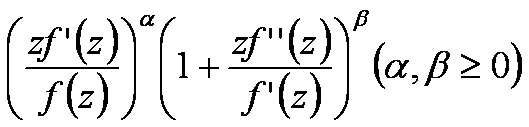lies in a region starlike with respect to 1 and symmetric with respect to the real axis. Also certain application of our main result for a class of functions defined by Hadamard product (convolution) is given. As a special case of our result we obtain Fekete-Szegö inequality for a class of functions defined through fractional derivatives. Also we obtain Fekete-Szegö inequality for the inverse functions.

1: Paper Source PDF document

Paper's Title:

Reverses of the Triangle Inequality in Inner Product Spaces

Author(s):

Sever S. Dragomir

School of Computer Science and Mathematics,
Victoria University Of Technology,
PO Box 14428, Mcmc 8001,
Victoria, Australia.
sever@csm.vu.edu.au
Url: http://rgmia.vu.edu.au/SSDragomirWeb.html

Abstract:

Some new reverses for the generalised triangle inequality in inner product spaces are given. Applications in connection to the Schwarz inequality and for vector-valued integrals are provided as well.

1: Paper Source PDF document

Paper's Title:

Positive Solution For Discrete Three-Point Boundary Value Problems

Author(s):

Wing-Sum Cheung And Jingli Ren

Department of Mathematics,
The University of Hong Kong,
Pokfulam, Hong Kong
wscheung@hku.hk

Institute of Systems Science,
Beijing 100080, P.R. China
renjl@mx.amss.ac.cn

Abstract:

This paper is concerned with the existence of positive solution to the discrete three-point boundary value problem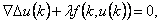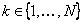,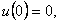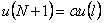where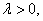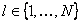, and f is allowed to change sign. By constructing available operators, we shall apply the method of lower solution and the method of topology degree to obtain positive solution of the above problem for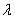on a suitable interval. The associated Green’s function is first given.

1: Paper Source PDF document

Paper's Title:

A Simple New Proof of Fan-Taussky-Todd Inequalities

Author(s):

Zhi-Hua Zhang and Zhen-Gang Xiao

Zixing Educational Research Section,
Chenzhou City, Hunan 423400, P. R. China.
Zhi-hua Zhang
Url: http://www.hnzxslzx.com/zzhweb/

Department Of Mathematics, Hunan Institute Of Science And Technology,
Yueyang City, Hunan 423400, P. R. China.
Zhen-gang Xiao

Abstract:

In this paper we present simple new proofs of the inequalities: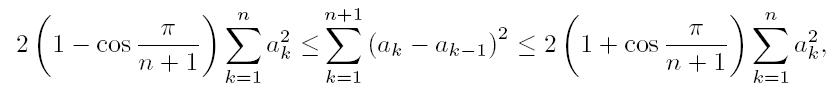which holds for all real numbers a0 = 0, a1, · · · , an, an+1 = 0 and the coefficients 2(1 - cos(π/(n + 1))) and 2(1 + cos(π/(n + 1))) are the best possible; and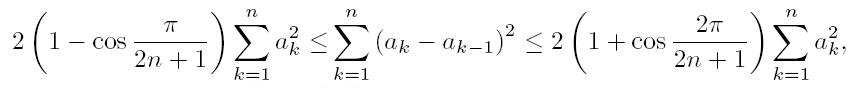which holds for all real numbers a0 = 0, a1, · · · , an and the coefficients 2(1-cos(π/(2n + 1))) and 2(1 + cos(π/(2n + 1))) are the best possible.

1: Paper Source PDF document

Paper's Title:

Weak Solution for Hyperbolic Equations with a Non-Local Condition

Author(s):

Lazhar Bougoffa

King Khalid University, Faculty of Science, Department of Mathematics,
P.O.Box 9004, Abha, Saudi Arabia

Abstract:

In this paper, we study hyperbolic equations with a non-local condition. We prove the existence and uniqueness of weak solutions, using energy inequality and the density of the range of the operator generated by the problem.

1: Paper Source PDF document

Paper's Title:

Meromorphic P-Valent Functions With Positive And Fixed Second Coefficients

Author(s):

B.A. Frasin and G. Murugusundaramoorthy

Department of Mathematics,
Al Al-Bayt University,
P.O. Box: 130095,
Mafraq, Jordan.
bafrasin@yahoo.com
URL: http://www.geocities.com/bafrasin/techie.html

Department of Mathematics,
Vellore Institute of Technology,
Deemed University,
Vellore - 632014,
India.
gmsmoorthy@yahoo.com

Abstract:

We introduce the classes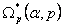and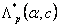of meromorphic univalent functions ith positive and fixed second coefficients. The aim of the present paper is to obtain coefficient inequalities and closure theorems for these classes. Furthermore, the radii of convexity and starlikeness for functions the classesandare determined.

1: Paper Source PDF document

Paper's Title:

The successive approximations method and error estimation in terms of at most the first derivative for delay ordinary differential equations

Author(s):

Alexandru Mihai Bica

Department of Mathematics,
Str. Armatei Romane no.5,
Romania
smbica@yahoo.com

Abstract:

We present here a numerical method for first order delay ordinary differential equations, which use the Banach's fixed point theorem, the sequence of successive approximations and the trapezoidal quadrature rule. The error estimation of the method uses a recent result of P. Cerone and S.S. Dragomir about the remainder of the trapezoidal quadrature rule for Lipchitzian functions and for functions with continuous first derivative.

1: Paper Source PDF document

Paper's Title:

On Zeros of Diagonally Quasiconvex Multifunctions

Author(s):

Zoran D. Mitrović

Faculty of Electrical Engineering,
University of Banja Luka,
78000 Banja Luka, Patre 5
Bosnia and Herzegovina
zmitrovic@etfbl.net

Abstract:

In this paper, we extended the notion of diagonally quasiconvexity for multifunctions and established several existence results for zeros of diagonally quasiconvex multifunctions. As applications we obtain the results of fixed points, coincidence points and best approximations for multifunctions. Using our result we also prove the existence of solutions to the variational-like inequality problem and generalized vector equilibrium problem. The results of this paper generalize some known results in the literature.

1: Paper Source PDF document

Paper's Title:

Positive Periodic Time-Scale Solutions for Functional Dynamic Equations

Author(s):

Douglas R. Anderson and Joan Hoffacker

Department of Mathematics and Computer Science
Concordia College
andersod@cord.edu
URL:
http://www.cord.edu/faculty/andersod/

Department of Mathematical Sciences
Clemson University
Clemson, SC 29634 USA
johoff@clemson.edu
URL:
http://www.math.clemson.edu/facstaff/johoff.htm

Abstract:

Using Krasnoselskii's fixed point theorem, we establish the existence of positive periodic solutions to two pairs of related nonautonomous functional delta dynamic equations on periodic time scales, and then extend the discussion to higher-dimensional equations. Two pairs of corresponding nabla equations are also provided in an analogous manner.

1: Paper Source PDF document

Paper's Title:

Notes on Sakaguchi Functions

Author(s):

Shigeyoshi Owa, Tadayuki Sekine and Rikuo Yamakawa

Department of Mathematics, Kinki University,
Higashi-Osaka, Osaka 577-8502,
Japan.
owa@math.kindai.ac.jp

Office of Mathematics, College of Pharmacy, Nihon University,
7-1 Narashinodai, Funabashi-city,
Chiba, 274-8555, Japan.
tsekine@pha.nihon-u.ac.jp

Department of Mathematics, Shibaura Institute of Technology,
Minuma, Saitama-city,
Saitama 337-8570, Japan.
yamakawa@sic.shibaura-it.ac.jp

Abstract:

By using the definition for certain univalent functions f(z) in the open unit disk U given by K. Sakaguchi , two classes S(α) and T(α) of analytic functions in U are introduced. The object of the present paper is to discuss some properties of functions f(z) belonging to the classes S(α) and T(α).

1: Paper Source PDF document

Paper's Title:

Boundary Value Problems for Fractional Diffusion-Wave equation

Author(s):

Varsha Daftardar-Gejji and Hossein Jafari

Department of Mathematics, University of Pune,
Ganeshkhind, Pune - 411007,
INDIA.
vsgejji@math.unipune.ernet.in
jafari_h@math.com

Abstract:

Non homogeneous fractional diffusion-wave equation has been solved under linear/nonlinear boundary conditions. As the order of time derivative changes from 0 to 2, the process changes from slow diffusion to classical diffusion to mixed diffusion-wave behaviour.
Numerical examples presented here confirm this inference. Orthogonality of eigenfunctions in case of fractional Stürm-Liouville problem has been established

1: Paper Source PDF document

Paper's Title:

Uniqueness of Meromorphic Functions that Share Three Values

Author(s):

Abhijit Banerjee

Department of Mathematics
Kalyani Government Engineering College
West Bengal 741235
India.
abanerjee_kal@yahoo.co.in
abanerjee@mail15.com
abanerjee_kal@rediffmail.com

Abstract:

In the paper dealing with the uniqueness problem of meromorphic functions we prove five theorems one of which will improve a result given by Lahiri \cite{5} and the remaining will supplement some previous results.

1: Paper Source PDF document

Paper's Title:

A Reverse of the Triangle Inequality in Inner Product Spaces and Applications for Polynomials

Author(s):

I. Brnetić, S. S. Dragomir, R. Hoxha and J. Pečarić

Department of Applied Mathematics, Faculty of Electrical Engineering and Computing,
University of Zagreb, Unska 3, 10 000 Zagreb,
Croatia
andrea@zpm.fer.hr

School of Computer Science & Mathematics, Victoria University
Po Box 14428, Melbourne Vic 8001
Australia
sever.dragomir@vu.edu.au
URL:http://rgmia.vu.edu.au/dragomir

Faculty of Applied Technical Sciences, University of Prishtina,
Mother Theresa 5, 38 000 Prishtina
Kosova
razimhoxha@yahoo.com

Faculty of Textile Technology, University of Zagreb,
Pierottijeva 6, 10000 Zagreb,
Croatia
pecaric@hazu.hr

Abstract:

A reverse of the triangle inequality in inner product spaces related to the celebrated Diaz-Metcalf inequality with applications for complex polynomials is given.

1: Paper Source PDF document

Paper's Title:

On the Fekete-Szegő Inequality for Some Subclasses of Analytic Functions

Author(s):

T.N. Shanmugam and A. Singaravelu

Department of Mathematics,
College of Engineering,
Anna University, Chennai-600 025,
shan@annauniv.edu

Department of Mathematics,
Valliammai Engineering College,
Chennai-603 203,
sivasaisastha@rediffmail.com

Abstract:

In this present investigation, the authors obtainFekete-Szegő's inequality for certain normalized analytic functions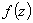defined on the open unit disk for which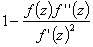lie in a region starlike with respect to 1 and symmetric with respect to the real axis. Also certain applications of the main result for a class of functions defined by convolution are given. As a special case of this result, Fekete-Szegő's inequality for a class of functions defined through fractional derivatives is also obtained.

1: Paper Source PDF document

Paper's Title:

Komatu Integral Transforms of Analytic Functions Subordinate to Convex Functions

Author(s):

T. N. Shanmugam and C. Ramachandran

Department of Mathematics, College of Engineering,
India
shan@annauniv.edu

Department of Mathematics, College of Engineering,
India
crjsp2004@yahoo.com

Abstract:

In this paper, we consider the class A of the functions f(z) of the form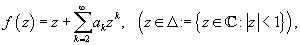which are analytic in an open disk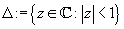and study certain subclass of the class A, for which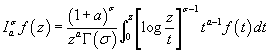has some property. Certain inclusion and the closure properties like convolution with convex univalent function etc. are studied.

1: Paper Source PDF document

Paper's Title:

A Coefficient Inequality For Certain Subclasses of Analytic Functions Related to Complex Order

Author(s):

B. Srutha Keerthi, B. Adolf Stephen and S. Sivasubramanian

Department Of Applied Mathematics, Sri Venkateswara College Of Engineering, Anna University,
Sriperumbudur, Chennai - 602 105,
India.
laya@svce.ac.in

Department of Mathematics, Madras Christian College, Chennai - 600059,
India

Department of Mathematics, College of Engineering, Anna University,
India.
sivasaisastha@rediffmail.com

Abstract:

In this present investigation, the authors obtain coefficient inequality for certain normalized analytic functions of complex order f(z) defined on the open unit disk for which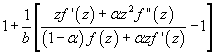(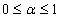and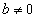be a complex number) lies in a region starlike with respect to 1 and is symmetric with respect to the real axis. Also certain applications of the main result for a class of functions of complex order defined by convolution are given. As a special case of this result, coefficient inequality for a class of functions defined through fractional derivatives is obtained. The motivation of this paper is to give a generalization of the coefficient inequalities of the subclasses of starlike and convex functions of complex order.

1: Paper Source PDF document

Paper's Title:

Construction of Lyapunov Functionals In Functional Differential Equations With Applications To Exponential Stability In Volterra Integro-differential Equations

Author(s):

Youssef N. Raffoul

Department of Mathematics, University of Dayton,
Dayton OH 45469-2316,
USA
youssef.raffoul@notes.udayton.edu

Abstract:

Non-negative definite Lyapunov functionals are employed to obtain sufficient conditions that guarantee the exponential asymptotic stability and uniform exponential asymptotic stability of the zero solution of nonlinear functional differential systems. The theory is applied to Volterra integro-differential equations in the form of proposition examples.

1: Paper Source PDF document

Paper's Title:

On Oscillation of Second-Order Delay Dynamic Equations on Time Scales

Author(s):

S. H. Saker

Department of Mathematics, Faculty of Science,
Mansoura University, Mansoura, 35516,
Egypt.
shsaker@mans.edu.eg

Abstract:

Some new oscillation criteria for second-order linear delay dynamic equation on a time scale T are established. Our results improve the recent results for delay dynamic equations and in the special case when T=R, the results include the oscillation results established by Hille [1948, Trans. Amer. Math. Soc. 64 (1948), 234-252] and Erbe [Canad. Math. Bull. 16 (1973), 49-56.] for differential equations. When T=Z the results include and improve some oscillation criteria for difference equations. When T=hZ, h>0, T=qN and T=N2, i.e., for generalized second order delay difference equations our results are essentially new and can be applied on different types of time scales. An example is considered to illustrate the main results.

1: Paper Source PDF document

Paper's Title:

Some Inequalities for a Certain Class of Multivalent Functions Using Multiplier Transformation

Author(s):

Department Of Applied Mathematics
Sri Venkateswara College Of Engineering
Sriperumbudur, Chennai - 602105,
India.
suchithravenkat@yahoo.co.in

Department Of Mathematics,
Chennai - 600059,
India.

Department Of Applied Mathematics
Sri Venkateswara College Of Engineering
Sriperumbudur, Chennai - 602105,
India.
ganga@svce.ac.in

Department Of Mathematics,
Easwari Engineering College
Ramapuram, Chennai - 600089,
India.
ganga@svce.ac.in

Abstract:

The object of the present paper is to derive several inequalities associated with differential subordinations between analytic functions and a linear operator defined for a certain family of p-valent functions, which is introduced here by means of a family of extended multiplier transformations. Some special cases and consequences of the main results are also considered.

1: Paper Source PDF document

Paper's Title:

Multivalent Harmonic Mappings Convoluted With a Multivalent Analytic Function

Author(s):

Om P. Ahuja and Özlem Güney

Kent State University, Department of Mathematical Sciences,
14111, Claridon-Troy Road, Burton, Ohio 44021,
U.S.A.
oahuja@kent.edu

University of Dicle, Department of Mathematics,
Faculty of Science and Art, 21280 Diyarbakir,
Turkey
ozlemg@dicle.edu.tr

Abstract:

The object of this paper is to study certain geometric properties of a family of multivalent harmonic mappings in the plane convoluted with a multivalent analytic function in the open unit disc.

1: Paper Source PDF document

Paper's Title:

A Subclass of Meromorphically Multivalent Functions with Applications to Generalized Hypergeometric Functions

Author(s):

M. K. Aouf

Mathematics Department, Faculty of Science,
Mansoura University 35516,
Egypt
mkaouf127@yahoo.com

Abstract:

In this paper a new subclass of meromorphically multivalent functions, which is defined by means of a Hadamard product (or convolution) involving some suitably normalized meromorphically p-valent functions. The main object of the present paper is to investigate the various important properties and characteristics of this subclass of meromorphically multivalent functions. We also derive many interesting results for the Hadamard products of functions belonging to this subclass. Also we consider several applications of our main results to generalized hypergeomtric functions.

1: Paper Source PDF document

Paper's Title:

Ulam Stability of Functional Equations

Author(s):

Stefan Czerwik and Krzysztof Król

Institute of Mathematics
Silesian University of Technology
Kaszubska 23, 44-100 Gliwice,
Poland

Stefan.Czerwik@polsl.pl
Krzysztof.Krol@polsl.pl

Abstract:

In this survey paper we present some of the main results on Ulam-Hyers-Rassias stability for important functional equations.

1: Paper Source PDF document

Paper's Title:

On the product of M-measures in l-groups

Author(s):

A. Boccuto, B. Riěcan, and A. R. Sambucini

Dipartimento di Matematica e Informatica,
via Vanvitelli, 1 I-06123 Perugia,
Italy.
boccuto@dipmat.unipg.it
URL: http://www.dipmat.unipg.it/~boccuto

Katedra Matematiky, Fakulta Prírodných Vied,
Univerzita Mateja Bela,
Tajovského, 40, Sk-97401 Banská Bystrica,
Slovakia.
riecan@fpv.umb.sk

Dipartimento di Matematica e Informatica,
via Vanvitelli, 1 I-06123 Perugia,
Italy.
matears1@unipg.it
URL: http://www.unipg.it/~matears1

Abstract:

Some extension-type theorems and compactness properties for the
product of l-group-valued M-measures are proved.

1: Paper Source PDF document

Paper's Title:

Asymptotic Distribution of Products of Weighted Sums of Dependent Random Variables

Author(s):

Y. Miao and J. F. Li

College of Mathematics and Information Science,
Henan Normal University
Henan, China
yumiao728@yahoo.com.cn

College of Mathematics and Information Science,
Henan Normal University, 453007
Henan, China.
junfen_li@yahoo.com.cn

Abstract:

In this paper we establish the asymptotic distribution of products of weighted sums of dependent positive random variable, which extends the results of Rempała and Wesołowski (2002).

1: Paper Source PDF document

Paper's Title:

Fractional Integral Operators and Olsen Inequalities on Non-Homogeneous Spaces

Author(s):

Idha Sihwaningrum, Herry P. Suryawan, Hendra Gunawan

Analysis and Geometry Group,
Faculty of Mathematics and Natural Sciences,
Bandung Institute of Technology, Bandung 40132,
Indonesia
hgunawan@math.itb.ac.id
URL: http://personal.fmipa.itb.ac.id/hgunawan/

Abstract:

We prove the boundedness of the fractional integral operator Iα on generalized Morrey spaces of non-homogeneous type. In addition, we also present Olsen-type inequalities for a multiplication operator involving Iα. Our proof uses a result of García-Cuerva and Martell .

1: Paper Source PDF document

Paper's Title:

Real Interpolation Methods and Quasilogarithmic Operators

Author(s):

Ming Fan

School of Industrial Technology and Management,
Dalarna University, 781 88 Borlänge, Sweden

fmi@du.se
URL: http://users.du.se/~fmi

Abstract:

The purpose of this paper is to deal with nonlinear quasilogarithmic operators, which possesses the uniformly bounded commutator property on various interpolation spaces in the sense of Brudnyi-Krugljak associated with the quasi-power parameter spaces. The duality, and the domain and range spaces of these operators are under consideration. Some known inequalities for the Lebesgue integration spaces and the trace classes are carried over to the non-commutative symmetric spaces of measurable operators affiliated with a semi-finite von Neumann algebra.

1: Paper Source PDF document

Paper's Title:

Hardy Type Inequalities via Convexity - The Journey so Far

Author(s):

James A. Oguntuase and Lars-Erik Persson

Department of Mathematics, University of Agriculture,
P. M. B. 2240, Abeokuta, Nigeria.

Department of Mathematics, Luleĺ University of Technology,
SE-971 87, Luleĺ , Sweden.

oguntuase@yahoo.com, larserik@sm.luth.se .

Abstract:

It is nowadays well-known that Hardy's inequality (like many other inequalities) follows directly from Jensen's inequality. Most of the development of Hardy type inequalities has not used this simple fact, which obviously was unknown by Hardy himself and many others. Here we report on some results obtained in this way mostly after 2002 by mainly using this fundamental idea.

1: Paper Source PDF document

Paper's Title:

On an Elliptic Over-Determined Problem in Dimension Two

Author(s):

Lakhdar Ragoub
Department of Mathematics and Information of Tiyadhechnology
AL Yamamah University
P.O. Box 45 180, Riyadh 11 512
Saudi Arabia.

lragoub@yu.edu.sa

Abstract:

We extend the method of Weinberger for a non-linear over-determined elliptic problem
in R2. We prove that the domain in consideration is a ball. The tool of this investigation are maximum principles and P-functions.

1: Paper Source PDF document

Paper's Title:

On Opial's Inequality for Functions of n-Independent Variables

Author(s):

S. A. A. El-Marouf and S. A. AL-Oufi

Department of Mathematics,
Faculty of Science,
Minoufiya University,
Shebin El-Koom,
Egypt

Department of Mathematics,
Faculty of Science, Taibah University,
Kingdom of Saudia Arabia

Abstract:

In this paper, we introduce Opial inequalities for functions of n-independent variables. Also, we discuss some different forms of Opial inequality containing functions of n independent variables and their partial derivatives with respect to independent variables.

1: Paper Source PDF document

Paper's Title:

Existence Results for Second Order Impulsive Functional Differential Equations with Infinite Delay

Author(s):

M. Lakrib, A. Oumansour and K. Yadi

Laboratoire de Mathématiques, Université Djillali
Liabées, B.P. 89 Sidi Bel Abbčs 22000, Algérie
mlakrib@univ-sba.dz
oumansour@univ-sba.dz

Laboratoire de Mathématiques, Université Abou Bekr
Belkaid, B.P. 119 Tlemcen 13000, Algérie

Abstract:

In this paper we study the existence of solutions for second order impulsive functional differential equations with infinite delay. To obtain our results, we apply fixed point methods.

1: Paper Source PDF document

Paper's Title:

Stability Problems for Generalized Additive Mappings and Euler-Lagrange Type Mappings

Author(s):

M. Todoroki, K. Kumahara, T. Miura and S.-E. Takahasi

The Open University of Japan,
Chiba, 261-8586,
Japan
tomamiyu3232@sky.sannet.ne.jp
kumahara@ouj.ac.jp

Yamagata University,
Yonezawa 992-8510,
Japan
miura@yz.yamagata-u.ac.jp

Toho University, Yamagata University,
Chiba, 273-0866,
Japan
sin_ei1@yahoo.co.jp

Abstract:

We introduce a generalized additivity of a mapping between Banach spaces and establish the Ulam type stability problem for a generalized additive mapping. The obtained results are somewhat different from the Ulam type stability result of Euler-Lagrange type mappings obtained by H. -M. Kim, K. -W. Jun and J. M. Rassias.

1: Paper Source PDF document

Paper's Title:

Szegö Limits and Haar Wavelet Basis

Author(s):

M. N. N. Namboodiri and S. Remadevi

Dept. of Mathematics, Cochin University of Science and Technology,
Cochin-21, Kerala,
India.

nambu@cusat.ac.in

Dept. of Mathematics, College of Engineering,
Cherthala, Kerala,
India.

rema@mec.ac.in

Abstract:

This paper deals with Szegö type limits for multiplication operators on L2 (R) with respect to Haar orthonormal basis. Similar studies have been carried out by Morrison for multiplication operators Tf using Walsh System and Legendre polynomials . Unlike the Walsh and Fourier basis functions, the Haar basis functions are local in nature. It is observed that Szegö type limit exist for a class of multiplication operators Tf , f∈ L (R) with respect to Haar (wavelet) system with appropriate ordering. More general classes of orderings of Haar system are identified for which the Szegö type limit exist for certain classes of multiplication operators. Some illustrative examples are also provided.

1: Paper Source PDF document

Paper's Title:

Some Identities for Ramanujan - Göllnitz - Gordon Continued fraction

Author(s):

M. S. Mahadeva Naika, B. N. Dharmendra and S. Chandan Kumar

Department of Mathematics,
Bangalore University,
Central College Campus,
Bangalore-560 001,
INDIA

msmnaika@rediffmail.com

chandan.s17@gmail.com

Department of Mathematics,
Maharani's Science College for Women,
J. L. B. Road, Mysore-570 001,
INDIA

dharmamath@rediffmail.com

Abstract:

In this paper, we obtain certain P--Q eta--function identities, using which we establish identities providing modular relations between Ramanujan-Göllnitz-Gordon continued fraction H(q) and H(q^n) for n= 2, 3, 4, 5, 7, 8, 9, 11, 13, 15, 17, 19, 23, 25, 29 and 55.

1: Paper Source PDF document

Paper's Title:

To a Banach *-algebra in a Semipartial Dynamical System

Author(s):

Bahman Tabatabaie Shourijeh and Seyed Mostafa Zebarjad

Department of Mathematics,
College of Sciences,
Shiraz University, Shiraz 71454,
Iran.

E-mail: tabataba@math.susc.ac.ir
URL: http://research.shirazu.ac.ir/faculty/More.asp?ID=207

Abstract:

By a partial dynamical system, we mean a triple containing a C*-algebra A, a discrete group G and a partial action of G on A. There are two C*--algebras associated to a given partial dynamical system. These are nothing but the certain C*-completions of a Banach *-algebra. In constructing such a Banach *-algebra, usually, a tedious limit process is used to apply. In this paper, we prove some theorems in this context without any limit process.

1: Paper Source PDF document

Paper's Title:

On Eigenvalues and Boundary Curvature of the C*-algebra Numerical Rang

Author(s):

M. T. Heydari

Department of Mathematics,
College of Sciences,
Yasouj University,
Yasouj, 75914-74831,
Iran.

E-mail: heydari@yu.ac.ir

Abstract:

Let A be a C*-algebra with unit 1 and a∈A be a nilpotent. By Donoghue's Theorem, all corner points of its numerical range V(a) belong to the spectrum σ(a). It is therefore natural to expect that, more generally, the distance from a point p on the boundary ∂ V(a) of V(a) to σ(a) should be in some sense bounded by the radius of curvature of ∂ V(a) at p.

1: Paper Source PDF document

Paper's Title:

The boundedness of Bessel-Riesz operators on generalized Morrey spaces

Author(s):

Mochammad Idris, Hendra Gunawan and Eridani

Department of Mathematics,
Bandung Institute of Technology,
Bandung 40132,
Indonesia.
E-mail: mochidris@students.itb.ac.id

Department of Mathematics,
Bandung Institute of Technology,
Bandung 40132,
Indonesia.
E-mail: hgunawan@math.itb.ac.id
URL: http://personal.fmipa.itb.ac.id/hgunawan/

Department of Mathematics,
Airlangga University,
Surabaya 60115,
Indonesia.

Abstract:

In this paper, we prove the boundedness of Bessel-Riesz operators on generalized Morrey spaces. The proof uses the usual dyadic decomposition, a Hedberg-type inequality for the operators, and the boundedness of Hardy-Littlewood maximal operator. Our results reveal that the norm of the operators is dominated by the norm of the kernels.

1: Paper Source PDF document

Paper's Title:

Stability of the D-Bar Reconstruction Method for Complex Conductivities

Author(s):

1S. El Kontar, 1T. El Arwadi, 1H. Chrayteh, 2J.-M. Sac-Épée

1Department of Mathematics and Computer Science,
Faculty of Science, Beirut Arab University,
P.O. Box: 11-5020, Beirut,
Lebanon.

E-mail: srs915@student.bau.edu.lb

2Institut Élie Cartan de Lorraine,
Université de Lorraine - Metz,
France.

Abstract:

In 2000, Francini solved the inverse conductivity problem for twice-differentiable conductivities and permittivities. This solution was considered to be the first approach using D-bar methods with complex conductivities. In 2012, based on Francini's work, Hamilton introduced a reconstruction method of the conductivity distribution with complex values. The method consists of six steps. A voltage potential is applied on the boundary. Solving a D-Bar equation gives the complex conductivity. In this paper, the stability of the D-Bar equation is studied via two approximations, texp and tB, for the scattering transform. The study is based on rewriting the reconstruction method in terms of continuous operators. The conductivity is considered to be non smooth.

1: Paper Source PDF document

Paper's Title:

A Note on Divergent Fourier Series and λ-Permutations

Author(s):

A. Castillo, J. Chavez and H. Kim

Tufts University,
Department of Mathematics,
Medford, MA 02155,
USA
E-mail: angel.castillo@tufts.edu

Texas Tech University,
Department of Mathematics and Statistics,
Lubbock, TX 79409,
USA
E-mail: josechavez5@my.unt.edu

University of Michigan-Dearborn,
Department of Mathematics and Statistics,
Dearborn, MI 48128,
USA.
E-mail: khyejin@umich.edu

Abstract:

We present a continuous function on [-π,π] whose Fourier series diverges and it cannot be rearranged to converge by a λ-permutation.

1: Paper Source PDF document

Paper's Title:

Existence of Positive Solutions for Nonlinear Fractional Differential Equations with Multi-point Boundary Conditions

Author(s):

Khenchela University, Department of Mathematics,
Khenchela, 40000,
Algeria.

Abstract:

This paper is devoted to the existence results of positive solutions for a nonlinear fractional differential equations with multi-point boundary conditions. By means of the Schauder fixed point theorem, some results on the existence are obtained.

1: Paper Source PDF document

Paper's Title:

Polyanalytic Functions on Subsets of Z[i]

Author(s):

Abtin Daghighi

SE-581 83,
Sweden.

E-mail: abtindaghighi@gmail.com

Abstract:

For positive integers q we consider the kernel of the powers Lq where L is one of three kinds of discrete analogues of the Cauchy-Riemann operator. The first two kinds are well-studied, but the third kind less so. We give motivations for further study of the third kind especially since its symmetry makes it more appealing for the cases q 2.

From an algebraic perspective it makes sense that the chosen multiplication on the kernels is compatible with the choice of pseudo-powers. We propose such multiplications together with associated pseudo-powers. We develop a proof-tool in terms of certain sets of uniqueness.

1: Paper Source PDF document

Paper's Title:

Fractional class of analytic functions Defined Using q-Differential Operator

Author(s):

K . R. Karthikeyan, Musthafa Ibrahim and S. Srinivasan

Department of Mathematics and Statistics,
Caledonian College of Engineering, Muscat,
Sultanate of Oman.
E-mail: kr_karthikeyan1979@yahoo.com

College of Engineering,
University of Buraimi, Al Buraimi,
Sultanate of Oman.
E-mail: musthafa.ibrahim@gmail.com

Department of Mathematics, Presidency College (Autonomous),
India.

Abstract:

We define a q-differential fractional operator, which generalizes Salagean and Ruscheweyh differential operators. We introduce and study a new class of analytic functions involving q-differential fractional operator. We also determine the necessary and sufficient conditions for functions to be in the class. Further, we obtain the coefficient estimates, extreme points, growth and distortion bounds.

1: Paper Source PDF document

Paper's Title:

On Commutator of Aluthge Transforms and Fuglede-Putnam Property

Author(s):

International Campus, Faculty of Mathematical Sciences,
Iran.
E-mail: manzar.maleki@gmail.com

Faculty of Mathematics and Statistics, Department of Mathematics,
University of Birjand,
P. O. Box 414, Birjand 9717851367,
Iran.

Department of Pure Mathematics, Hakim Sabzevari University,
P.O. Box 397, Sabzevar,
Iran.

Abstract:

We deal with the well-known Fuglede-Putnam theorem and related FP-property. We show that if (A,B) has the FP-property, then so has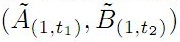where 0 t1,t21 are arbitrary. We first prove that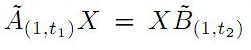if and only if AX=XB for all X, whenever (A,B) has the FP-property. We prove some similar results for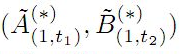instead of \$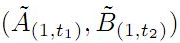as well. Also we introduce the sequence of generalized iterations of Aluthge transform of operators and express some results for this notion associated to the FP-property.

1: Paper Source PDF document

Paper's Title:

An Analytical Solution of Perturbed Fisher's Equation Using Homotopy Perturbation Method (HPM), Regular Perturbation Method (RPM) and Adomian Decomposition Method (ADM)

Author(s):

Moussa Bagayogo, Youssouf Minoungou, Youssouf Pare

Departement de Mathematique,
Universite Ouaga I Pr Joseph Ki-Zerbo,
Burkina Faso.
E-mail: moussabagayogo94@gmail.com, m.youl@yahoo.fr, pareyoussouf@yahoo.fr.

Abstract:

In this paper, Homotopy Perturbation Method (HPM), Regular Pertubation Method (RPM) and Adomian decomposition Method (ADM) are applied to Fisher equation. Then, the solution yielding the given initial conditions is gained. Finally, the solutions obtained by each method are compared

1: Paper Source PDF document

Paper's Title:

Dynamical Analysis of HIV/AIDS Epidemic Model with Two Latent Stages, Vertical Transmission and Treatment

Author(s):

Nur Shofianah, Isnani Darti, Syaiful Anam

Mathematics Department,Faculty of Mathematics and Natural Sciences.
University of Brawijaya,
Jl. Veteran, Malang 65145,
Indonesia.
E-mail: nur_shofianah@ub.ac.id, isnanidarti@ub.ac.id, syaiful@ub.ac.id

Abstract:

We discuss about dynamical analysis of HIV/AIDS epidemic model with two latent stages, vertical transmission and treatment. In this model, the spreading of HIV occurs through both horizontal and vertical transmission. There is also treatment for individual who has been HIV infected. The latent stage is divided into slow and fast latent stage based on the immune condition which varies for each individual. Dynamical analysis result shows that the model has two equilibrium points: the disease-free equilibrium point and the endemic equilibrium point. The existence and global stability of equilibrium points depend on the basic reproduction number R0. When R0 <1, only the disease-free equilibrium point exists. If R0 >1, there are two equilibrium points, which are the disease-free equilibrium point and the endemic equilibrium point. Based on the result of stability analysis, the disease-free equilibrium point is globally asymptotically stable if R0 <1, while if R0 > 1 and p=q, the endemic equilibrium point will be globally asymptotically stable. In the end, we show some numerical simulations to support the analytical result.

1: Paper Source PDF document

Paper's Title:

An Existence of the Solution to Neutral Stochastic Functional Differential Equations Under the Holder Condition

Author(s):

Young-Ho Kim

Department of Mathematics,
Changwon National University,
Changwon, Gyeongsangnam-do 51140,
Korea.

E-mail: iyhkim@changwon.ac.kr

Abstract:

In this paper, we show the existence and uniqueness of solution of the neutral stochastic functional differential equations under weakened H\"{o}lder condition, a weakened linear growth condition, and a contractive condition. Furthermore, in order to obtain the existence of a solution to the equation we used the Picard sequence.

1: Paper Source PDF document

Paper's Title:

Weyl's theorem for class Q and k - quasi class Q Operators

Author(s):

S. Parvatham and D. Senthilkumar

Department of Mathematics and Humanities,
Sri Ramakrishna Institute of Technology, Coimbatore-10, Tamilnadu,
India.
E-mail: parvathasathish@gmail.com

Post Graduate and Research Department of Mathematics,
India.
E-mail: senthilsenkumhari@gmail.com

Abstract:

In this paper, we give some properties of class  Q  operators. It is proved that every class  Q  operators satisfies Weyl's theorem under the condition that  T2  is isometry. Also we proved that every  k  quasi class  Q  operators is Polaroid and the spectral mapping theorem holds for this class of operator. It will be proved that single valued extension property, Weyl and generalized Weyl's theorem holds for every  k  quasi class  Q  operators.

1: Paper Source PDF document

Paper's Title:

On Ruled Surfaces According to Quasi-Frame in Euclidean 3-Space

Author(s):

M. Khalifa Saad and R. A. Abdel-Baky

Department of Mathematics, Faculty of Science,
KSA.
Department of Mathematics, Faculty of Science,
Sohag University, Sohag,
EGYPT.
E-mail: mohamed_khalifa77@science.sohag.edu.eg, mohammed.khalifa@iu.edu.sa

Department of Mathematics, Faculty of Science,
Assiut University, Assiut,
EGYPT.
E-mail: rbaky@live.com

Abstract:

This paper aims to study the skew ruled surfaces by using the quasi-frame of Smarandache curves in the Euclidean 3-space. Also, we reveal the relationship between Serret-Frenet and quasi-frames and give a parametric representation of a directional ruled surface using the quasi-frame. Besides, some comparative examples are given and plotted which support our method and main results.

1: Paper Source PDF document

Paper's Title:

Antiderivatives and Integrals Involving Incomplete Beta Functions with Applications

Author(s):

Mathematics Department,
Yarmouk University,
Irbid 21163,
Jordan.
E-mail: rami_thenat@yu.edu.jo

Faculty of Engineering,
Higher Colleges of Technology,
Ras Alkhaimah,
UAE.

Abstract:

In this paper, we prove that incomplete beta functions are antiderivatives of several products and powers of trigonometric functions, we give formulas for antiderivatives for products and powers of trigonometric functions in term of incomplete beta functions, and we evaluate integrals involving trigonometric functions using incomplete beta functions. Also, we extend some properties of the beta functions to the incomplete beta functions. As an application for the above results, we find the moments for certain probability distributions.

1: Paper Source PDF document

Paper's Title:

Reduced Generalized Combination Synchronization Between Two n-Dimensional Integer-Order Hyperchaotic Systems and One m-Dimensional Fractional-Order Chaotic System

Author(s):

Smail Kaouache, Mohammed Salah Abdelouahab and Rabah Bououden

Laboratory of Mathematics and their interactions,
Abdelhafid Boussouf University Center, Mila.
Algeria
E-mail: smailkaouache@gmail.com, medsalah3@yahoo.fr, rabouden@yahoo.fr

Abstract:

This paper is devoted to investigate the problem of reduced generalized combination synchronization (RGCS) between two n-dimensional integer-order hyperchaotic drive systems and one m-dimensional fractional-order chaotic response system. According to the stability theorem of fractional-order linear system, an active mode controller is proposed to accomplish this end. Moreover, the proposed synchronization scheme is applied to synchronize three different chaotic systems, which are the Danca hyperchaotic system, the modified hyperchaotic Rossler system, and the fractional-order Rabinovich-Fabrikant chaotic system. Finally, numerical results are presented to fit our theoretical analysis.

1: Paper Source PDF document

Paper's Title:

Existence and Approximation of Traveling Wavefronts for the Diffusive Mackey-Glass Equation

Author(s):

C. Ramirez-Carrasco and J. Molina-Garay

Chile
E-mail: carloshrc1989@gmail.com
molina@imca.edu.pe

Abstract:

In this paper, we consider the diffusive Mackey-Glass model with discrete delay. This equation describes the dynamics of the blood cell production. We investigate the existence of traveling wavefronts solutions connecting the two steady states of the model. We develop an alternative proof of the existence of such solutions and we also demonstrate the existence of traveling wavefronts moving at minimum speed. The proposed approach is based on the use technique of upper-lower solutions. Finally, through an iterative procedure, we show numerical simulations that approximate the traveling wavefronts, thus confirming our theoretical results.

1: Paper Source PDF document

Paper's Title:

Coexisting Attractors and Bubbling Route to Chaos in Modified Coupled Duffing Oscillators

Author(s):

B. Deruni1, A. S. Hacinliyan1,2, E. Kandiran3, A. C. Keles2, S. Kaouache4, M.-S. Abdelouahab4, N.-E. Hamri4

1Department of Physics,
University of Yeditepe,
Turkey.

2Department of Information Systems and Technologies,
University of Yeditepe,
Turkey

3Department of Software Development,
University of Yeditepe,
Turkey.

4Laboratory of Mathematics and their interactions,
University Center of Abdelhafid Boussouf,
Mila 43000,
Algeria.

E-mail: berc890@gmail.com
ahacinliyan@yeditepe.edu.tr
engin.kandiran@yeditepe.edu.tr
cihan.keles@yeditepe.edu.tr
s.kaouache@centr-univ-mila.dz
medsalah3@yahoo.fr
n.hamri@centre-univ-mila.dz

Abstract:

In this article dynamical behavior of coupled Duffing oscillators is analyzed under a small modification. The oscillators have cubic damping instead of linear one. Although single duffing oscillator has complex dynamics, coupled duffing systems possess a much more complex structure. The dynamical behavior of the system is investigated both numerically and analytically. Numerical results indicate that the system has double scroll attractor with suitable parameter values. On the other hand, bifurcation diagrams illustrate rich behavior of the system, and it is seen that, system enters into chaos with different routes. Beside classical bifurcations, bubbling route to chaos is observed for suitable parameter settings. On the other hand, Multistability of the system is indicated with the coexisting attractors, such that under same parameter setting the system shows different periodic and chaotic attractors. Moreover, chaotic synchronization of coupled oscillators is illustrated in final section.

1: Paper Source PDF document

Paper's Title:

Pointwise Convergence of Fourier-type Series with Exponential Weights

Author(s):

Hee Sun Jung and Ryozi Sakai

Department of Mathematics Education, Sungkyunkwan University,
Seoul 110-745,
Republic of Korea.
E-mail: hsun90@skku.edu

Department of Mathematics,
Meijo University, Nagoya 468-8502,
Japan.
E-mail: ryozi@hm.aitai.ne.jp

Abstract:

Let R = ( - ∞,∞), and let Q∈C1(R):R→[0,∞) be an even function. We consider the exponential weights w(x)=e-Q(x), xR. In this paper we obtain a pointwise convergence theorem for the Fourier-type series with respect to the orthonormal polynomials {pn(w2;x)}.

1: Paper Source PDF document

Paper's Title:

On Euler's First Transformation Formula for k-hypergeometric Function

Author(s):

Sungtae Jun and Insuk Kim

General Education Institute,
Konkuk University, Chungju 380-701,
Republic of Korea.
E-mail: sjun@kku.ac.kr

Department of Mathematics Education,
Wonkwang University, Iksan, 570-749,
Republic of Korea.
E-mail: iki@wku.ac.kr

Abstract:

Mubeen et al. obtained Kummer's first transformation for the k-hypergeometric function. The aim of this note is to provide the Euler-type first transformation for the k-hypergeometric function. As a limiting case, we recover the results of Mubeen et al. In addition to this, an alternate and easy derivation of Kummer's first transformation for the k-hypergeometric function is also given.

1: Paper Source PDF document

Paper's Title:

Hankel Functional Connected to Lemniscate of Bernoulli

Author(s):

K. Ramanuja Rao, Rajnesh Lal and Kaushal Singh

Fiji National University,
Department of Mathematics & Statistics,
P.O. Box 5529, Lautoka,
Fiji.
E-mail: ramanuja.kotti@fnu.ac.fj
rajnesh.lal@fnu.ac.fj
kaushal.singh@fnu.ac.fj

Abstract:

The aim of present paper is to derive a higher bound (HB) of 3rd order Hankel determinant for a collection of holomorphic mappings connected with exactly to the right side of the lemniscate of Bernoulli, whose polar coordinates form is r2 = 2cos2(2θ). The method carried in this paper is more refined than the method adopted by the authors (see ), who worked on this problem earlier.

1: Paper Source PDF document

Paper's Title:

Locally Bicomplex Convex Module and Their Applications

Author(s):

Department of Mathematics,
University of Jammu,
Jammu And Kashmir,
India.
E-mail: stanzinkunga19@gmail.com

Department of Mathematics,
University of Jammu,
Jammu And Kashmir,
India.

Abstract:

Let X be a locally BC convex module and L(X) be the family of all continuous bicomplex linear operators on X. In this paper, we study some concepts of D-valued seminorms on locally BC convex module. Further, we study the bicomplex version of Co and (Co,1) semigroup. The work of this paper is inspired by the work in  and .

1: Paper Source PDF document

Paper's Title:

Algorithms for Nonlinear Problems Involving Strictly Pseudocontractive Mappings

Author(s):

Mathew Olajiire Aibinu1, Surendra Colin Thakur2, Sibusiso Moyo3

1Institute for Systems Science & KZN E-Skill CoLab,
Durban University of Technology,
Durban 4000,
South Africa.

1DSI-NRF Centre of Excellence in Mathematical and Statistical Sciences (CoE-MaSS),
Johannesburg,
South Africa.
E-mail:
moaibinu@yahoo.com mathewa@dut.ac.za

2 KZN E-Skill CoLab,
Durban University of Technology,
Durban 4000,
South Africa.
E-mail:
thakur@dut.ac.za

3Institute for Systems Science & Office of the DVC Research, Innovation & Engagement Milena Court,
Durban University of Technology,
Durban 4000,
South Africa.
E-mail:
dvcrie@dut.ac.za

Abstract:

The puzzles in approximating a fixed point of nonlinear problems involving the class of strictly pseudocontractive mappings are conquered in this paper through viscosity implicit rules. Using generalized contraction mappings, a new viscosity iterative algorithm which is implicit in nature is proposed and analysed in Banach spaces for the class of strictly pseudocontractive mappings. The computations and analysis which are used in the proposed scheme are easy to follow and this gives rooms for a broad application of the scheme. It is obtained that the proposed iterative algorithm converges strongly to a fixed point of a μ-strictly pseudocontractive mapping which also solves a variational inequality problem. The result is also shown to hold for finite family of strictly pseudocontractive mappings. A numerical example is given to show the skillfulness of the proposed scheme and its implementation.

1: Paper Source PDF document

Paper's Title:

A Review on Minimally Supported Frequency Wavelets

Author(s):

K Pallavi1, M C Lineesh1, A Noufal2

1Department of Mathematics,
National Institute of Technology Calicut,
Kerala 673601,
India.
E-mail:
pavikrishnakumar@gmail.com
lineesh@nitc.ac.in

2Department of Mathematics,
Cochin University of Science and Technology,
Kerala 682022,
India.
E-mail: noufal@cusat.ac.in

Abstract:

This paper provides a review on Minimally Supported Frequency (MSF) wavelets that includes the construction and characterization of MSF wavelets. The characterization of MSF wavelets induced from an MRA is discussed and the nature of the low-pass filter associated with it is explained. The concept of wavelet set and dimension function is introduced to study this class of wavelets. Along with MSF wavelets, s-elementary wavelets and unimodular wavelets are also considered due to the similarity in definitions. Examples and illustrations are provided for more clarity.

1: Paper Source PDF document

Paper's Title:

On General Class of Nonlinear Contractive Maps and their Performance Estimates

Author(s):

Olalekan Taofeek Wahab and Salaudeen Alaro Musa

Department of Mathematics and Statistics
Kwara State University, Malete
P. M. B. 1530 Ilorin,
Nigeria.
E-mail: taofeek.wahab@kwasu.edu.ng

Abstract:

This paper considers two independent general class of nonlinear contractive maps to study the existence properties of nonlinear operators with prior degenerate. The existence properties are proved in the framework of approximate fixed points with the imposition of the general class of contractive conditions in metrical convex spaces without emphasis on completeness or compactness. For computational purposes, the performance estimates and the sensitivity dependence of these conditions are obtained for the Picard operator. Practical examples are also considered to justify the validity of the conditions. The results ensure no term is lost in the operators with prior degenerate and the conditions are strictly larger class when compare with others in the literature.

1: Paper Source PDF document

Paper's Title:

On Trigonometric Approximation of Continuous Functions by Deferred Matrix Means

Author(s):

Xhevat Zahir Krasniqi

Faculty of Education,
University of Prishtina "Hasan Prishtina",
Avenue "Mother Theresa " no. 5, Prishtin
ë 10000,
Republic of Kosovo.
E-mail: xhevat.krasniqi@uni-pr.edu
URL: https://staff.uni-pr.edu/profile/xhevatkrasniqi

Abstract:

In this paper, for the first time, we introduce the deferred matrix means which contain the well-known generalized deferred Nörlund, deferred Nörlund, deferred Riesz, deferred Cesŕro means introduced earlier by others, and a new class of sequences (predominantly a wider class than the class of Head Bounded Variation Sequences). In addition, using the deferred matrix means of Fourier series of a continuous function, we determine the degree of approximation of such function via its modulus of continuity and a positive mediate function.

1: Paper Source PDF document

Paper's Title:

Three Inequalities Associated with Rado Inequality

Author(s):

Rin Miyao, Yusuke Nishizawa, Keigo Takamura

Faculty of Education,
Saitama University,
Shimo-okubo 255, Sakura-ku, Saitama-city, Saitama,
Japan.
E-mail: r.miyao.242@ms.saitama-u.ac.jp

Faculty of Education,
Saitama University,
Shimo-okubo 255, Sakura-ku, Saitama-city, Saitama,
Japan.
E-mail: ynishizawa@mail.saitama-u.ac.jp

Faculty of Education,
Saitama University,
Shimo-okubo 255, Sakura-ku, Saitama-city, Saitama,
Japan.
E-mail: k.takamura.442@ms.saitama-u.ac.jp

Abstract:

In this short note we estimate three inequalities associated with Rado inequality and show the refinement and reverse of Arithmetic mean- Geometric mean inequality.

1: Paper Source PDF document

Paper's Title:

Several New Closed-form Evaluations of the Generalized Hypergeometric Function with Argument 1/16

Author(s):

B. R. Srivatsa Kumar, Insuk Kim and Arjun K. Rathie

Department of Mathematics,
Manipal Institute of Technology,
Manipal 576 104,
India.
E-mail: sri_vatsabr@yahoo.com

Department of Mathematics Education,
Wonkwang University,
Iksan, 54538,
Republic of Korea.
E-mail: iki@wku.ac.kr

Department of Mathematics,
Vedant College of Engineering and Technology,
Rajasthan Technical University,
Bundi, 323021, Rajasthan,
India.
E-mail: arjunkumarrathie@gmail.com

Abstract:

The main objective of this paper is to establish as many as thirty new closed-form evaluations of the generalized hypergeometric function q+1Fq(z) for q= 2, 3, 4. This is achieved by means of separating the generalized hypergeometric function q+1Fq(z) for q=1, 2, 3, 4, 5 into even and odd components together with the use of several known infinite series involving central binomial coefficients obtained earlier by Ji and Hei \& Ji and Zhang.

1: Paper Source PDF document

Paper's Title:

Application of Chebyshev Polynomials to Volterra-Fredholm Integral Equations

Author(s):

Department of Mathematics,
Faculty of Mathematics and
Informatics,
University of Msila,
Algeria.

E-mail: aissa.lakhal@univ-msila.dz

Abstract:

The goal of this work is to examine the numerical solution of linear Volterra-Fredholm integral equations of the second kind using the first, second, third and fourth Chebyshev polynomials. Noting that, the approximate solution is given in the form of series which converges to the exact one. Numerical examples are compared with other methods, in order to prove the applicability and the efficiency of this technical.

1: Paper Source PDF document

Paper's Title:

Fractional Integral Inequalities of Hermite-Hadamard Type for P-convex and Quasi-Convex Stochastic Process

Author(s):

Oualid Rholam, Mohammed Barmaki and Driss Gretet

National School of Applied Sciences (ENSA),
University Ibn Tofail,
B.P 242 Kenitra 14000,
phone number : +212606257757,
Morocco.
E-mail: oualid.rholam@uit.ac.ma

Science Faculty Ben M'sik,
University Hassan II,
B.P 7955 Av Driss El Harti Sidi Othmane 20700,
phone number : +212 5 22 70 46 71 ,
Morocco.
E-mail:  mohammed.barmaki@uit.ac.ma

National School of Applied Sciences (ENSA),
University Ibn Tofail,
B.P 242 Kenitra 14000,
phone number : +212661403557,
Morocco.
E-mail: driss.gretete@uit.ac.ma

Abstract:

In this paper we consider the class of P-convex and Quasi-convex stochastic processes on witch we apply a general class of generalized fractional integral operator in order to establish new integral inequalities of Hermite-Hadammard type. then we obtain some results for well known types of fractional integrals. Results obtained in this paper may be starting point as well as a useful source of inspiration for further research in convex analysis.

1: Paper Source PDF document

Paper's Title:

Complete Analysis of Global Behavior of Certain System of Piecewise Linear Difference Equations

Author(s):

Atiratch Laoharenoo, Ratinan Boonklurb and Watcharapol Rewlirdsirikul

Department of Mathematics and Computer Science,
Thailand.
E-mail: atiratch.l@kvis.ac.th

Department of Mathematics and Computer Science,
Faculty of Science, Chulalongkorn University, Bangkok 10330
Thailand.
E-mail: ratinan.b@chula.ac.th

Department of Mathematics and Computer Science,
Faculty of Science, Chulalongkorn University, Bangkok 10330
Thailand.
E-mail: 6570104323@student.chula.ac.th

Abstract:

Our goal is to study the system of piecewise linear difference equations x{n+1} = |xn|-yn-b and y{n+1} = xn - |yn| + 1 where n 0 and b6. We can prove that the behavior of the solution can be divided into 2 types depending on the region of initial condition (x0,y0). That is, the solution eventually becomes the equilibrium point. Otherwise, the solution eventually becomes the periodic solution of prime period 5. All regions of initial condition for each type of solution are determined.

Search and serve lasted 0 second(s).